# 13 Diabetic Monitoring

The following example will show-case one method processing data from strings and processing into dates and factors.

The folder Diabetes-Data is referenced from <https://archive.ics.uci.edu/ml/datasets/Diabetes>. as per the website:

## Diabetes Data Set

Abstract: This diabetes dataset is from AIM ’94

 Data Set Characteristics: Multivariate, Time-Series Number of Instances: ? Area: Life Attribute Characteristics: Categorical, Integer Number of Attributes: 20 Date Donated Not Reported Associated Tasks: Missing Values? ? Number of Web Hits: 561559 as reported on of April 2021

Source: Michael Kahn, MD, PhD, Washington University, St. Louis, MO

Data Set Information: Diabetes patient records were obtained from two sources: an automatic electronic recording device and paper records. The automatic device had an internal clock to timestamp events, whereas the paper records only provided “logical time” slots (breakfast, lunch, dinner, bedtime). For paper records, fixed times were assigned to breakfast (08:00), lunch (12:00), dinner (18:00), and bedtime (22:00). Thus paper records have fictitious uniform recording times whereas electronic records have more realistic time stamps.

Diabetes files consist of four fields per record. Each field is separated by a tab and each record is separated by a newline.

File format:

1. Date in MM-DD-YYYY format
2. Time in XX:YY format
3. Code
4. Value

The Code field is deciphered as follows:

• 33 = Regular insulin dose
• 34 = NPH insulin dose
• 35 = UltraLente insulin dose
• 48 = Unspecified blood glucose measurement
• 57 = Unspecified blood glucose measurement
• 58 = Pre-breakfast blood glucose measurement
• 59 = Post-breakfast blood glucose measurement
• 60 = Pre-lunch blood glucose measurement
• 61 = Post-lunch blood glucose measurement
• 62 = Pre-supper blood glucose measurement
• 63 = Post-supper blood glucose measurement
• 64 = Pre-snack blood glucose measurement
• 65 = Hypoglycemic symptoms
• 66 = Typical meal ingestion
• 67 = More-than-usual meal ingestion
• 68 = Less-than-usual meal ingestion
• 69 = Typical exercise activity
• 70 = More-than-usual exercise activity
• 71 = Less-than-usual exercise activity
• 72 = Unspecified special event

$$~$$

$$~$$

Week_6_wd <-  file.path('C:/Users/jkyle/Documents/GitHub/Jeff_Data_Wrangling/Week_6')
Week_6_DATA <- file.path(Week_6_wd,"DATA")
Week_6_Funs <- file.path(Week_6_wd,"FUNCTIONS")

DM2_DATA <- file.path(Week_6_wd,'Diabetes-Data')
DM2_DATA
#>  "C:/Users/jkyle/Documents/GitHub/Jeff_Data_Wrangling/Week_6/Diabetes-Data"
list_of_file_paths <- list.files(path = DM2_DATA,
pattern = 'data-',
recursive = TRUE,
full.names = TRUE)
list_of_file_paths
#>   "C:/Users/jkyle/Documents/GitHub/Jeff_Data_Wrangling/Week_6/Diabetes-Data/data-01"
#>   "C:/Users/jkyle/Documents/GitHub/Jeff_Data_Wrangling/Week_6/Diabetes-Data/data-02"
#>   "C:/Users/jkyle/Documents/GitHub/Jeff_Data_Wrangling/Week_6/Diabetes-Data/data-03"
#>   "C:/Users/jkyle/Documents/GitHub/Jeff_Data_Wrangling/Week_6/Diabetes-Data/data-04"
#>   "C:/Users/jkyle/Documents/GitHub/Jeff_Data_Wrangling/Week_6/Diabetes-Data/data-05"
#>   "C:/Users/jkyle/Documents/GitHub/Jeff_Data_Wrangling/Week_6/Diabetes-Data/data-06"
#>   "C:/Users/jkyle/Documents/GitHub/Jeff_Data_Wrangling/Week_6/Diabetes-Data/data-07"
#>   "C:/Users/jkyle/Documents/GitHub/Jeff_Data_Wrangling/Week_6/Diabetes-Data/data-08"
#>   "C:/Users/jkyle/Documents/GitHub/Jeff_Data_Wrangling/Week_6/Diabetes-Data/data-09"
#>  "C:/Users/jkyle/Documents/GitHub/Jeff_Data_Wrangling/Week_6/Diabetes-Data/data-10"
#>  "C:/Users/jkyle/Documents/GitHub/Jeff_Data_Wrangling/Week_6/Diabetes-Data/data-11"
#>  "C:/Users/jkyle/Documents/GitHub/Jeff_Data_Wrangling/Week_6/Diabetes-Data/data-12"
#>  "C:/Users/jkyle/Documents/GitHub/Jeff_Data_Wrangling/Week_6/Diabetes-Data/data-13"
#>  "C:/Users/jkyle/Documents/GitHub/Jeff_Data_Wrangling/Week_6/Diabetes-Data/data-14"
#>  "C:/Users/jkyle/Documents/GitHub/Jeff_Data_Wrangling/Week_6/Diabetes-Data/data-15"
#>  "C:/Users/jkyle/Documents/GitHub/Jeff_Data_Wrangling/Week_6/Diabetes-Data/data-16"
#>  "C:/Users/jkyle/Documents/GitHub/Jeff_Data_Wrangling/Week_6/Diabetes-Data/data-17"
#>  "C:/Users/jkyle/Documents/GitHub/Jeff_Data_Wrangling/Week_6/Diabetes-Data/data-18"
#>  "C:/Users/jkyle/Documents/GitHub/Jeff_Data_Wrangling/Week_6/Diabetes-Data/data-19"
#>  "C:/Users/jkyle/Documents/GitHub/Jeff_Data_Wrangling/Week_6/Diabetes-Data/data-20"
#>  "C:/Users/jkyle/Documents/GitHub/Jeff_Data_Wrangling/Week_6/Diabetes-Data/data-21"
#>  "C:/Users/jkyle/Documents/GitHub/Jeff_Data_Wrangling/Week_6/Diabetes-Data/data-22"
#>  "C:/Users/jkyle/Documents/GitHub/Jeff_Data_Wrangling/Week_6/Diabetes-Data/data-23"
#>  "C:/Users/jkyle/Documents/GitHub/Jeff_Data_Wrangling/Week_6/Diabetes-Data/data-24"
#>  "C:/Users/jkyle/Documents/GitHub/Jeff_Data_Wrangling/Week_6/Diabetes-Data/data-25"
#>  "C:/Users/jkyle/Documents/GitHub/Jeff_Data_Wrangling/Week_6/Diabetes-Data/data-26"
#>  "C:/Users/jkyle/Documents/GitHub/Jeff_Data_Wrangling/Week_6/Diabetes-Data/data-27"
#>  "C:/Users/jkyle/Documents/GitHub/Jeff_Data_Wrangling/Week_6/Diabetes-Data/data-28"
#>  "C:/Users/jkyle/Documents/GitHub/Jeff_Data_Wrangling/Week_6/Diabetes-Data/data-29"
#>  "C:/Users/jkyle/Documents/GitHub/Jeff_Data_Wrangling/Week_6/Diabetes-Data/data-30"
#>  "C:/Users/jkyle/Documents/GitHub/Jeff_Data_Wrangling/Week_6/Diabetes-Data/data-31"
#>  "C:/Users/jkyle/Documents/GitHub/Jeff_Data_Wrangling/Week_6/Diabetes-Data/data-32"
#>  "C:/Users/jkyle/Documents/GitHub/Jeff_Data_Wrangling/Week_6/Diabetes-Data/data-33"
#>  "C:/Users/jkyle/Documents/GitHub/Jeff_Data_Wrangling/Week_6/Diabetes-Data/data-34"
#>  "C:/Users/jkyle/Documents/GitHub/Jeff_Data_Wrangling/Week_6/Diabetes-Data/data-35"
#>  "C:/Users/jkyle/Documents/GitHub/Jeff_Data_Wrangling/Week_6/Diabetes-Data/data-36"
#>  "C:/Users/jkyle/Documents/GitHub/Jeff_Data_Wrangling/Week_6/Diabetes-Data/data-37"
#>  "C:/Users/jkyle/Documents/GitHub/Jeff_Data_Wrangling/Week_6/Diabetes-Data/data-38"
#>  "C:/Users/jkyle/Documents/GitHub/Jeff_Data_Wrangling/Week_6/Diabetes-Data/data-39"
#>  "C:/Users/jkyle/Documents/GitHub/Jeff_Data_Wrangling/Week_6/Diabetes-Data/data-40"
#>  "C:/Users/jkyle/Documents/GitHub/Jeff_Data_Wrangling/Week_6/Diabetes-Data/data-41"
#>  "C:/Users/jkyle/Documents/GitHub/Jeff_Data_Wrangling/Week_6/Diabetes-Data/data-42"
#>  "C:/Users/jkyle/Documents/GitHub/Jeff_Data_Wrangling/Week_6/Diabetes-Data/data-43"
#>  "C:/Users/jkyle/Documents/GitHub/Jeff_Data_Wrangling/Week_6/Diabetes-Data/data-44"
#>  "C:/Users/jkyle/Documents/GitHub/Jeff_Data_Wrangling/Week_6/Diabetes-Data/data-45"
#>  "C:/Users/jkyle/Documents/GitHub/Jeff_Data_Wrangling/Week_6/Diabetes-Data/data-46"
#>  "C:/Users/jkyle/Documents/GitHub/Jeff_Data_Wrangling/Week_6/Diabetes-Data/data-47"
#>  "C:/Users/jkyle/Documents/GitHub/Jeff_Data_Wrangling/Week_6/Diabetes-Data/data-48"
#>  "C:/Users/jkyle/Documents/GitHub/Jeff_Data_Wrangling/Week_6/Diabetes-Data/data-49"
#>  "C:/Users/jkyle/Documents/GitHub/Jeff_Data_Wrangling/Week_6/Diabetes-Data/data-50"
#>  "C:/Users/jkyle/Documents/GitHub/Jeff_Data_Wrangling/Week_6/Diabetes-Data/data-51"
#>  "C:/Users/jkyle/Documents/GitHub/Jeff_Data_Wrangling/Week_6/Diabetes-Data/data-52"
#>  "C:/Users/jkyle/Documents/GitHub/Jeff_Data_Wrangling/Week_6/Diabetes-Data/data-53"
#>  "C:/Users/jkyle/Documents/GitHub/Jeff_Data_Wrangling/Week_6/Diabetes-Data/data-54"
#>  "C:/Users/jkyle/Documents/GitHub/Jeff_Data_Wrangling/Week_6/Diabetes-Data/data-55"
#>  "C:/Users/jkyle/Documents/GitHub/Jeff_Data_Wrangling/Week_6/Diabetes-Data/data-56"
#>  "C:/Users/jkyle/Documents/GitHub/Jeff_Data_Wrangling/Week_6/Diabetes-Data/data-57"
#>  "C:/Users/jkyle/Documents/GitHub/Jeff_Data_Wrangling/Week_6/Diabetes-Data/data-58"
#>  "C:/Users/jkyle/Documents/GitHub/Jeff_Data_Wrangling/Week_6/Diabetes-Data/data-59"
#>  "C:/Users/jkyle/Documents/GitHub/Jeff_Data_Wrangling/Week_6/Diabetes-Data/data-60"
#>  "C:/Users/jkyle/Documents/GitHub/Jeff_Data_Wrangling/Week_6/Diabetes-Data/data-61"
#>  "C:/Users/jkyle/Documents/GitHub/Jeff_Data_Wrangling/Week_6/Diabetes-Data/data-62"
#>  "C:/Users/jkyle/Documents/GitHub/Jeff_Data_Wrangling/Week_6/Diabetes-Data/data-63"
#>  "C:/Users/jkyle/Documents/GitHub/Jeff_Data_Wrangling/Week_6/Diabetes-Data/data-64"
#>  "C:/Users/jkyle/Documents/GitHub/Jeff_Data_Wrangling/Week_6/Diabetes-Data/data-65"
#>  "C:/Users/jkyle/Documents/GitHub/Jeff_Data_Wrangling/Week_6/Diabetes-Data/data-66"
#>  "C:/Users/jkyle/Documents/GitHub/Jeff_Data_Wrangling/Week_6/Diabetes-Data/data-67"
#>  "C:/Users/jkyle/Documents/GitHub/Jeff_Data_Wrangling/Week_6/Diabetes-Data/data-68"
#>  "C:/Users/jkyle/Documents/GitHub/Jeff_Data_Wrangling/Week_6/Diabetes-Data/data-69"
#>  "C:/Users/jkyle/Documents/GitHub/Jeff_Data_Wrangling/Week_6/Diabetes-Data/data-70"
library('tidyverse')
#> -- Attaching packages --------------------------------------- tidyverse 1.3.1 --
#> v ggplot2 3.3.3     v purrr   0.3.4
#> v tibble  3.1.2     v dplyr   1.0.6
#> v tidyr   1.1.3     v stringr 1.4.0
#> v readr   1.4.0     v forcats 0.5.1
#> -- Conflicts ------------------------------------------ tidyverse_conflicts() --

tibble_of_file_paths <- tibble(list_of_file_paths) %>%
mutate(full_path = list_of_file_paths) %>%
mutate(file_no = str_replace(string = full_path,
pattern = paste0(DM2_DATA,'/data-'),
replacement = ''))

tibble_of_file_paths
#> # A tibble: 70 x 3
#>   list_of_file_paths                    full_path                        file_no
#>   <chr>                                 <chr>                            <chr>
#> 1 C:/Users/jkyle/Documents/GitHub/Jeff~ C:/Users/jkyle/Documents/GitHub~ 01
#> 2 C:/Users/jkyle/Documents/GitHub/Jeff~ C:/Users/jkyle/Documents/GitHub~ 02
#> 3 C:/Users/jkyle/Documents/GitHub/Jeff~ C:/Users/jkyle/Documents/GitHub~ 03
#> 4 C:/Users/jkyle/Documents/GitHub/Jeff~ C:/Users/jkyle/Documents/GitHub~ 04
#> 5 C:/Users/jkyle/Documents/GitHub/Jeff~ C:/Users/jkyle/Documents/GitHub~ 05
#> 6 C:/Users/jkyle/Documents/GitHub/Jeff~ C:/Users/jkyle/Documents/GitHub~ 06
#> # ... with 64 more rows
read_in_text_from_file_no <- function(my_file_no){

enquo_by <- enquo(my_file_no)

cur_file <- tibble_of_file_paths %>%
filter(file_no == !!enquo_by)

cur_path <- as.character(cur_file$full_path) dat <- readr::read_tsv(cur_path, col_names = c('Date',"Time","Code","Value"), col_types = cols(col_character(), col_character(), col_character(), col_character()) ) %>% mutate(file_no = as.numeric(cur_file$file_no))

return(dat)
}
library('furrr')
no_cores <- availableCores() - 1
no_cores
#> system
#>      3

future::plan(multicore, workers = no_cores)
DM2_TIME_RAW <- furrr::future_map_dfr(tibble_of_file_paths$file_no, # list of columns function(x){ read_in_text_from_file_no(x) }) DM2_TIME_RAW %>% saveRDS('C:/Users/jkyle/Documents/GitHub/Jeff_Data_Wrangling/DATA/DM2_TIME_RAW.RDS') DM2_TIME_RAW <- readRDS('C:/Users/jkyle/Documents/GitHub/Jeff_Data_Wrangling/DATA/DM2_TIME_RAW.RDS') #> -- Attaching packages --------------------------------------- tidyverse 1.3.1 -- #> v ggplot2 3.3.3 v purrr 0.3.4 #> v tibble 3.1.2 v dplyr 1.0.6 #> v tidyr 1.1.3 v stringr 1.4.0 #> v readr 1.4.0 v forcats 0.5.1 #> -- Conflicts ------------------------------------------ tidyverse_conflicts() -- #> x dplyr::filter() masks stats::filter() #> x dplyr::lag() masks stats::lag() $$~$$ $$~$$ ## 13.2 Decode Table Code_Table <- tribble( ~ Code, ~ Code_Description, 33 , "Regular insulin dose", 34 , "NPH insulin dose", 35 , "UltraLente insulin dose", 48 , "Unspecified blood glucose measurement", # This decode value is the same as 57 57 , "Unspecified blood glucose measurement", # This decode value is the same as 48 58 , "Pre-breakfast blood glucose measurement", 59 , "Post-breakfast blood glucose measurement", 60 , "Pre-lunch blood glucose measurement", 61 , "Post-lunch blood glucose measurement", 62 , "Pre-supper blood glucose measurement", 63 , "Post-supper blood glucose measurement", 64 , "Pre-snack blood glucose measurement", 65 , "Hypoglycemic symptoms", 66 , "Typical meal ingestion", 67 , "More-than-usual meal ingestion", 68 , "Less-than-usual meal ingestion", 69 , "Typical exercise activity", 70 , "More-than-usual exercise activity", 71 , "Less-than-usual exercise activity", 72 , "Unspecified special event") %>% # What does this mean? mutate(Code_Invalid = if_else(Code %in% c(48,57,72), TRUE, FALSE)) %>% mutate(Code_New = if_else(Code_Invalid == TRUE, 999, Code)) Code_Table #> # A tibble: 20 x 4 #> Code Code_Description Code_Invalid Code_New #> <dbl> <chr> <lgl> <dbl> #> 1 33 Regular insulin dose FALSE 33 #> 2 34 NPH insulin dose FALSE 34 #> 3 35 UltraLente insulin dose FALSE 35 #> 4 48 Unspecified blood glucose measurement TRUE 999 #> 5 57 Unspecified blood glucose measurement TRUE 999 #> 6 58 Pre-breakfast blood glucose measurement FALSE 58 #> # ... with 14 more rows Code_Table_Factor <- Code_Table %>% filter(Code_Invalid == FALSE) %>% mutate(Code_Factor = factor(paste0("C_",Code_New))) Code_Table_Factor #> # A tibble: 17 x 5 #> Code Code_Description Code_Invalid Code_New Code_Factor #> <dbl> <chr> <lgl> <dbl> <fct> #> 1 33 Regular insulin dose FALSE 33 C_33 #> 2 34 NPH insulin dose FALSE 34 C_34 #> 3 35 UltraLente insulin dose FALSE 35 C_35 #> 4 58 Pre-breakfast blood glucose measureme~ FALSE 58 C_58 #> 5 59 Post-breakfast blood glucose measurem~ FALSE 59 C_59 #> 6 60 Pre-lunch blood glucose measurement FALSE 60 C_60 #> # ... with 11 more rows ## 13.3 Format Data library('lubridate') #> #> Attaching package: 'lubridate' #> The following objects are masked from 'package:base': #> #> date, intersect, setdiff, union DM2_TIME <- DM2_TIME_RAW %>% mutate(Date_Time_Char = paste0(Date, ' ', Time)) %>% mutate(Date_Time = mdy_hm(Date_Time_Char)) %>% mutate(Value_Num = as.numeric(Value)) %>% mutate(Code = as.numeric(Code)) %>% mutate(Record_ID = row_number()) #> Warning: 45 failed to parse. #> Warning in mask$eval_all_mutate(quo): NAs introduced by coercion

DM2_TIME
#> # A tibble: 29,330 x 9
#>   Date    Time   Code Value file_no Date_Time_Char Date_Time           Value_Num
#>   <chr>   <chr> <dbl> <chr>   <dbl> <chr>          <dttm>                  <dbl>
#> 1 04-21-~ 9:09     58 100         1 04-21-1991 9:~ 1991-04-21 09:09:00       100
#> 2 04-21-~ 9:09     33 009         1 04-21-1991 9:~ 1991-04-21 09:09:00         9
#> 3 04-21-~ 9:09     34 013         1 04-21-1991 9:~ 1991-04-21 09:09:00        13
#> 4 04-21-~ 17:08    62 119         1 04-21-1991 17~ 1991-04-21 17:08:00       119
#> 5 04-21-~ 17:08    33 007         1 04-21-1991 17~ 1991-04-21 17:08:00         7
#> 6 04-21-~ 22:51    48 123         1 04-21-1991 22~ 1991-04-21 22:51:00       123
#> # ... with 29,324 more rows, and 1 more variable: Record_ID <int>
UnKnown_Measurements <- DM2_TIME %>%
left_join(Code_Table_Factor, by='Code') %>%
filter(is.na(Code_Description) | is.na(Date_Time) | is.na(Value_Num) | Code_Invalid == TRUE | Code_New == 999 )

UnKnown_Measurements
#> # A tibble: 3,171 x 13
#>   Date    Time   Code Value file_no Date_Time_Char Date_Time           Value_Num
#>   <chr>   <chr> <dbl> <chr>   <dbl> <chr>          <dttm>                  <dbl>
#> 1 04-21-~ 22:51    48 123         1 04-21-1991 22~ 1991-04-21 22:51:00       123
#> 2 04-24-~ 22:09    48 340         1 04-24-1991 22~ 1991-04-24 22:09:00       340
#> 3 04-25-~ 21:54    48 288         1 04-25-1991 21~ 1991-04-25 21:54:00       288
#> 4 04-28-~ 22:30    48 200         1 04-28-1991 22~ 1991-04-28 22:30:00       200
#> 5 04-29-~ 22:28    48 081         1 04-29-1991 22~ 1991-04-29 22:28:00        81
#> 6 04-30-~ 23:06    48 104         1 04-30-1991 23~ 1991-04-30 23:06:00       104
#> # ... with 3,165 more rows, and 5 more variables: Record_ID <int>,
#> #   Code_Description <chr>, Code_Invalid <lgl>, Code_New <dbl>,
#> #   Code_Factor <fct>
Known_Measurements <- DM2_TIME %>%
anti_join(UnKnown_Measurements %>% select(Record_ID)) %>%
left_join(Code_Table_Factor, by='Code')
#> Joining, by = "Record_ID"

Known_Measurements
#> # A tibble: 26,159 x 13
#>   Date    Time   Code Value file_no Date_Time_Char Date_Time           Value_Num
#>   <chr>   <chr> <dbl> <chr>   <dbl> <chr>          <dttm>                  <dbl>
#> 1 04-21-~ 9:09     58 100         1 04-21-1991 9:~ 1991-04-21 09:09:00       100
#> 2 04-21-~ 9:09     33 009         1 04-21-1991 9:~ 1991-04-21 09:09:00         9
#> 3 04-21-~ 9:09     34 013         1 04-21-1991 9:~ 1991-04-21 09:09:00        13
#> 4 04-21-~ 17:08    62 119         1 04-21-1991 17~ 1991-04-21 17:08:00       119
#> 5 04-21-~ 17:08    33 007         1 04-21-1991 17~ 1991-04-21 17:08:00         7
#> 6 04-22-~ 7:35     58 216         1 04-22-1991 7:~ 1991-04-22 07:35:00       216
#> # ... with 26,153 more rows, and 5 more variables: Record_ID <int>,
#> #   Code_Description <chr>, Code_Invalid <lgl>, Code_New <dbl>,
#> #   Code_Factor <fct>

$$~$$

$$~$$

## 13.4 View Data

Known_Measurements %>%
ggplot(aes(x=Date_Time, y=Value_Num, color=Code_Factor)) +
geom_point()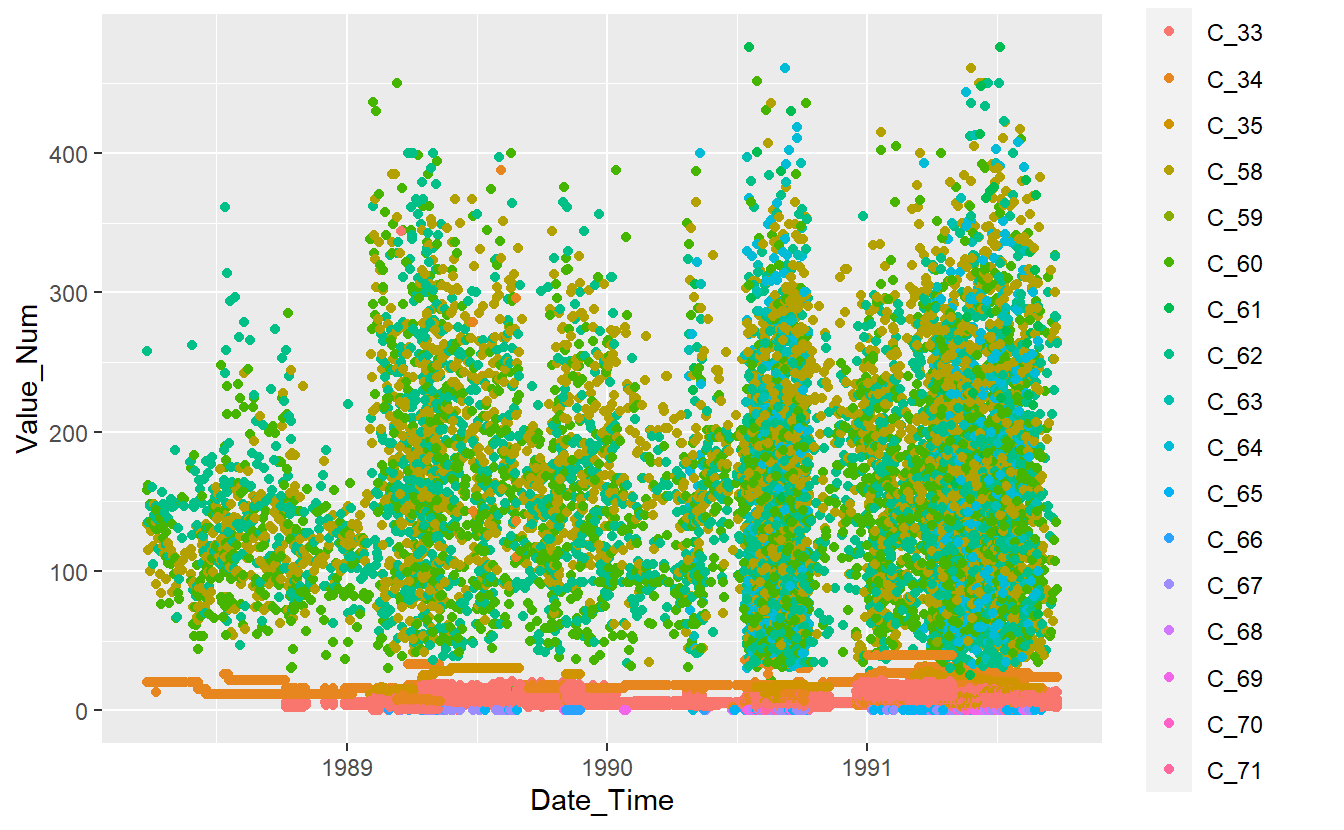Features_Percent_Complete <- function(data, Percent_Complete = 0){

SumNa <- function(col){sum(is.na(col))}

na_sums <- data %>%
summarise_all(SumNa) %>%
tidyr::pivot_longer(everything() ,names_to = 'feature', values_to = 'SumNa') %>%
arrange(-SumNa) %>%
mutate(PctNa = SumNa/nrow(data)) %>%
mutate(PctComp = (1 - PctNa)*100)

data_out <- na_sums %>%
filter(PctComp >= Percent_Complete)

return(data_out)
}
Feature_Percent_Complete_Graph <- function(data, Percent_Complete = 0 ){
table <- Features_Percent_Complete(data, Percent_Complete)

plot1 <- table %>%
ggplot(aes(x=reorder(feature, PctComp), y =PctComp, fill=feature)) +
geom_bar(stat = "identity") +
coord_flip() +
theme(legend.position = "none")
return(plot1)
} 
Feature_Percent_Complete_Graph(data = Known_Measurements %>% select(Date_Time, Value_Num, Code_Factor) )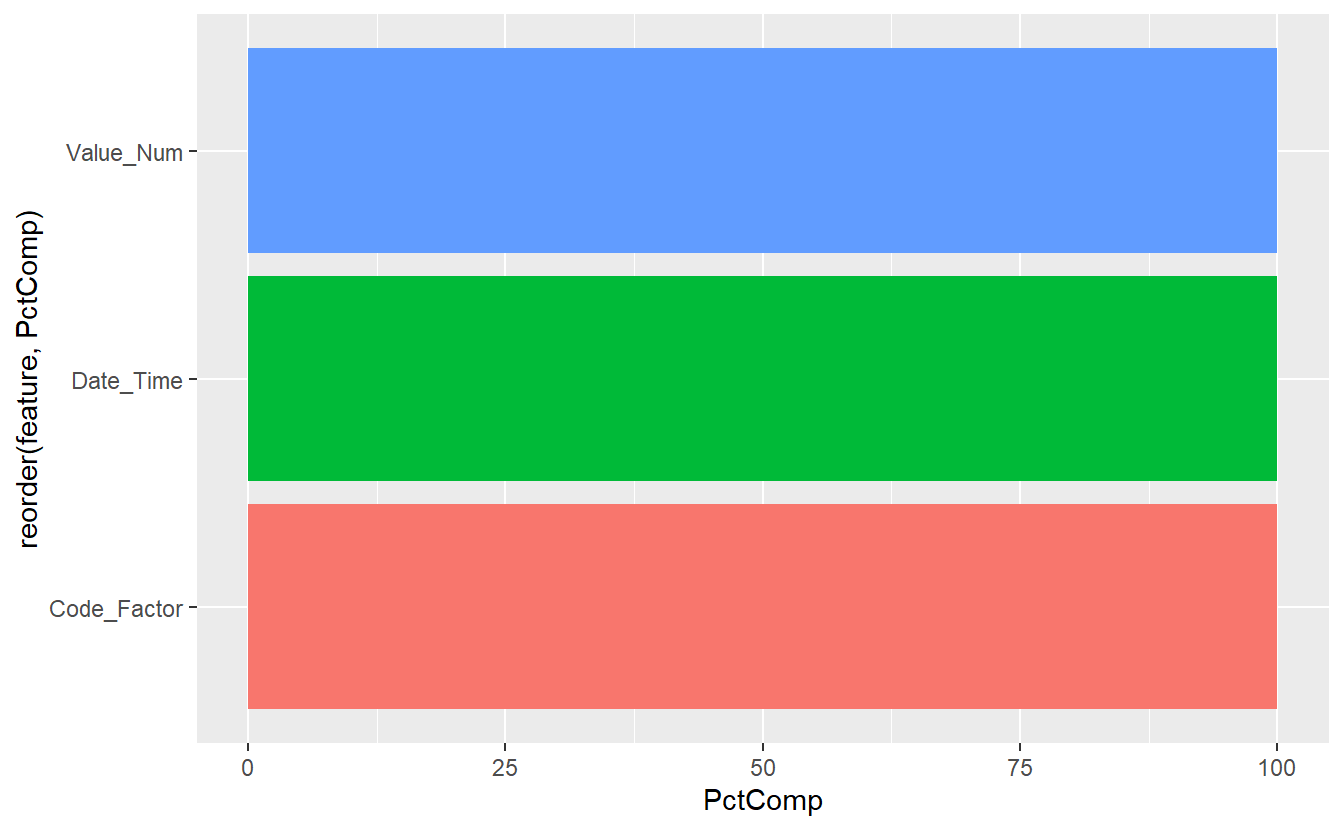Known Measurements Feature % Complete Graph

$$~$$

$$~$$

## 13.5 UnKnown Measurements

UnKnown_Measurements %>%
ggplot(aes(x=Date_Time, y=Value_Num)) +
geom_point()
#> Warning: Removed 86 rows containing missing values (geom_point).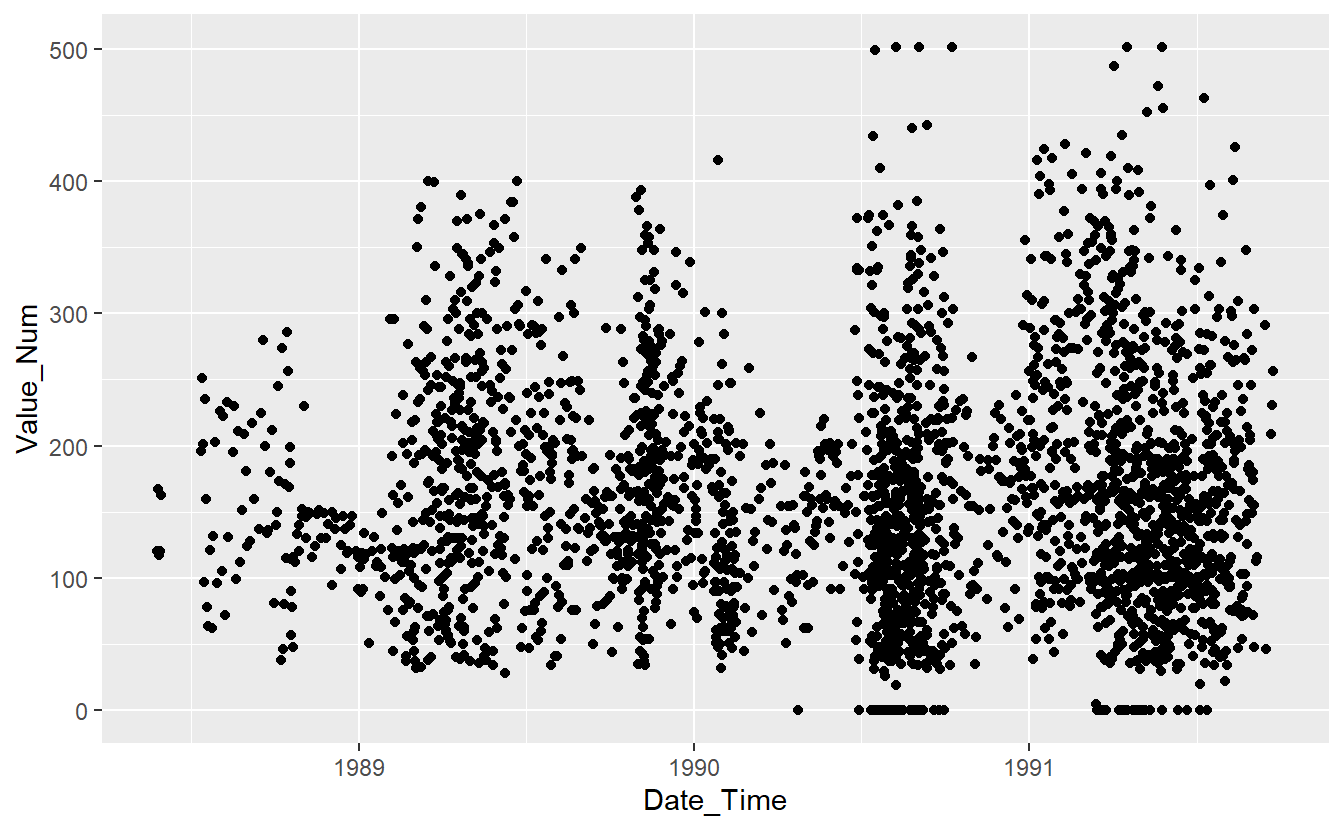UnKnown Measurements

$$~$$

$$~$$

## 13.6 Split Training Data

set.seed(123)
train_records <- sample(Known_Measurements$Record_ID, size = nrow(Known_Measurements)*.6, replace = FALSE) DATA.train <- Known_Measurements %>% filter(Record_ID %in% train_records) DATA.test <- Known_Measurements %>% filter(!(Record_ID %in% train_records)) DATA.train %>% ggplot(aes(x=Date_Time, y=Value_Num, color=Code_Factor)) + geom_point()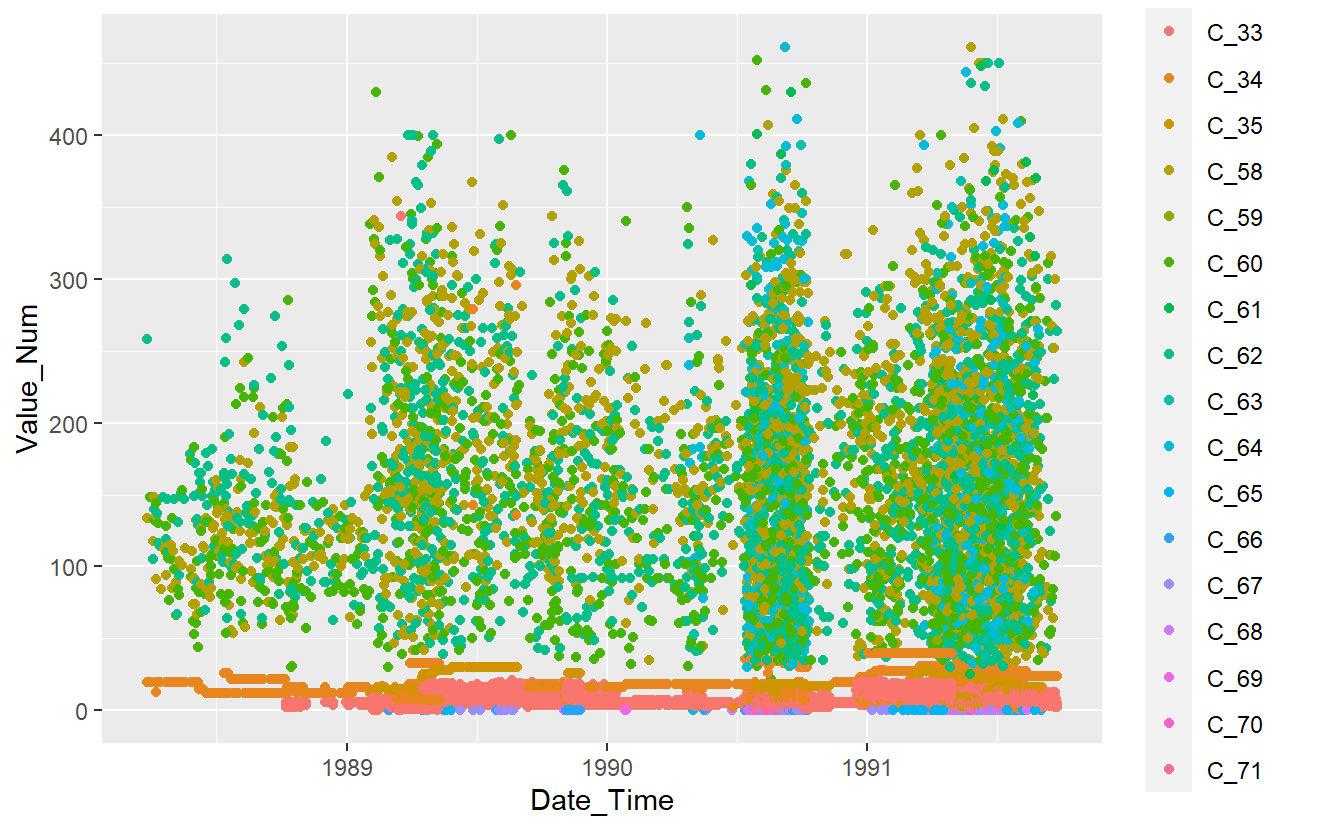Plot of Training Data DATA.test %>% ggplot(aes(x=Date_Time, y=Value_Num, color=Code_Factor)) + geom_point()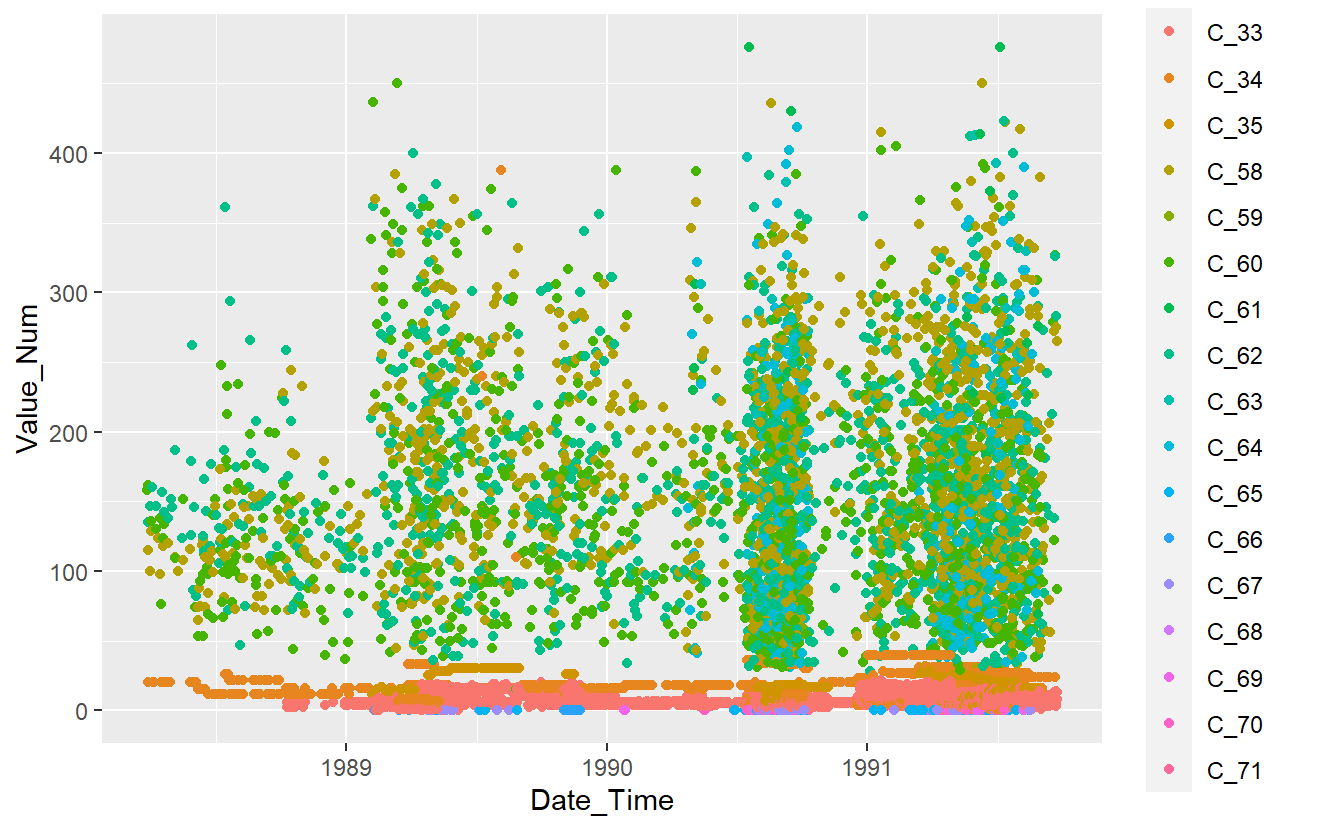Plot of Test Data $$~$$ $$~$$ ## 13.7 Fit a Model library('randomForest') #> randomForest 4.6-14 #> Type rfNews() to see new features/changes/bug fixes. #> #> Attaching package: 'randomForest' #> The following object is masked from 'package:dplyr': #> #> combine #> The following object is masked from 'package:ggplot2': #> #> margin rf_model <- randomForest(Code_Factor ~ Date_Time + Value_Num, data = DATA.train, ntree = 659, importance = TRUE) ### 13.7.1 Random Forest Ouput Here’s the output of Random Forest rf_model #> #> Call: #> randomForest(formula = Code_Factor ~ Date_Time + Value_Num, data = DATA.train, ntree = 659, importance = TRUE) #> Type of random forest: classification #> Number of trees: 659 #> No. of variables tried at each split: 1 #> #> OOB estimate of error rate: 36.11% #> Confusion matrix: #> C_33 C_34 C_35 C_58 C_59 C_60 C_61 C_62 C_63 C_64 C_65 C_66 C_67 C_68 C_69 #> C_33 5377 231 59 0 0 0 0 2 0 0 1 1 3 0 0 #> C_34 669 1586 59 4 0 2 0 1 0 0 0 0 0 0 0 #> C_35 167 88 398 0 0 0 0 0 0 0 0 0 0 0 0 #> C_58 0 5 3 969 1 473 6 551 21 79 0 0 0 0 0 #> C_59 0 0 0 3 0 3 0 5 0 0 0 0 0 0 0 #> C_60 0 10 1 555 2 534 1 465 9 66 0 0 0 0 0 #> C_61 0 0 1 12 0 7 4 12 0 4 0 0 0 0 0 #> C_62 0 8 0 651 2 440 1 680 10 85 0 0 0 0 0 #> C_63 0 0 0 45 0 25 0 35 26 5 0 0 0 0 0 #> C_64 0 5 0 137 0 85 0 127 7 173 0 1 0 0 0 #> C_65 0 0 0 0 0 0 0 0 0 0 47 4 143 0 0 #> C_66 1 0 0 0 0 0 0 0 0 0 3 66 27 0 0 #> C_67 1 0 0 0 0 0 0 0 0 0 32 1 167 0 0 #> C_68 0 0 0 0 0 0 0 0 0 0 3 2 16 0 0 #> C_69 0 0 0 0 0 0 0 0 0 0 3 11 25 0 0 #> C_70 0 0 0 0 0 0 0 0 0 0 4 1 85 0 0 #> C_71 0 0 0 0 0 0 0 0 0 0 8 0 47 0 0 #> C_70 C_71 class.error #> C_33 0 0 0.05234403 #> C_34 0 0 0.31667385 #> C_35 0 0 0.39050536 #> C_58 0 0 0.54032258 #> C_59 0 0 1.00000000 #> C_60 0 0 0.67498478 #> C_61 0 0 0.90000000 #> C_62 0 0 0.63771977 #> C_63 0 0 0.80882353 #> C_64 0 0 0.67663551 #> C_65 0 0 0.75773196 #> C_66 0 0 0.31958763 #> C_67 0 0 0.16915423 #> C_68 0 0 1.00000000 #> C_69 0 0 1.00000000 #> C_70 0 0 1.00000000 #> C_71 0 0 1.00000000 The Confusion Matrix that we see above is the confusion matrix from fitting the model against the training data so it is overly optimistic in terms of predictions. Random Forest is a collection of Decision Trees, we can look at the individual trees in the forest by using one of the two following functions. library(ggraph) library(igraph) #> #> Attaching package: 'igraph' #> The following objects are masked from 'package:lubridate': #> #> %--%, union #> The following objects are masked from 'package:future': #> #> %->%, %<-% #> The following objects are masked from 'package:dplyr': #> #> as_data_frame, groups, union #> The following objects are masked from 'package:purrr': #> #> compose, simplify #> The following object is masked from 'package:tidyr': #> #> crossing #> The following object is masked from 'package:tibble': #> #> as_data_frame #> The following objects are masked from 'package:stats': #> #> decompose, spectrum #> The following object is masked from 'package:base': #> #> union plot_rf_tree <- function(final_model, tree_num, shorten_label = TRUE) { # get tree by index tree <- randomForest::getTree(final_model, k = tree_num, labelVar = TRUE) %>% tibble::rownames_to_column() %>% # make leaf split points to NA, so the 0s won't get plotted mutate(split point = ifelse(is.na(prediction), split point, NA)) # prepare data frame for graph graph_frame <- data.frame(from = rep(tree$rowname, 2),
to = c(tree$left daughter, tree$right daughter))

# convert to graph and delete the last node that we don't want to plot
graph <- graph_from_data_frame(graph_frame) %>%
delete_vertices("0")

# set node labels
V(graph)$node_label <- gsub("_", " ", as.character(tree$split var))

if (shorten_label) {
V(graph)$leaf_label <- substr(as.character(tree$prediction), 1, 1)
}

V(graph)$split <- as.character(round(tree$split point, digits = 2))

# plot
plot <- ggraph(graph, 'tree') +
theme_graph() +
geom_node_point() +
geom_node_label(aes(label = leaf_label, fill = leaf_label), na.rm = TRUE,
repel = FALSE, colour = "white",
show.legend = FALSE)

print(plot)
}
plot_rf_tree(rf_model, 456)
#> Warning: Duplicated aesthetics after name standardisation: na.rm
#> Warning: Removed 2855 rows containing missing values (geom_label).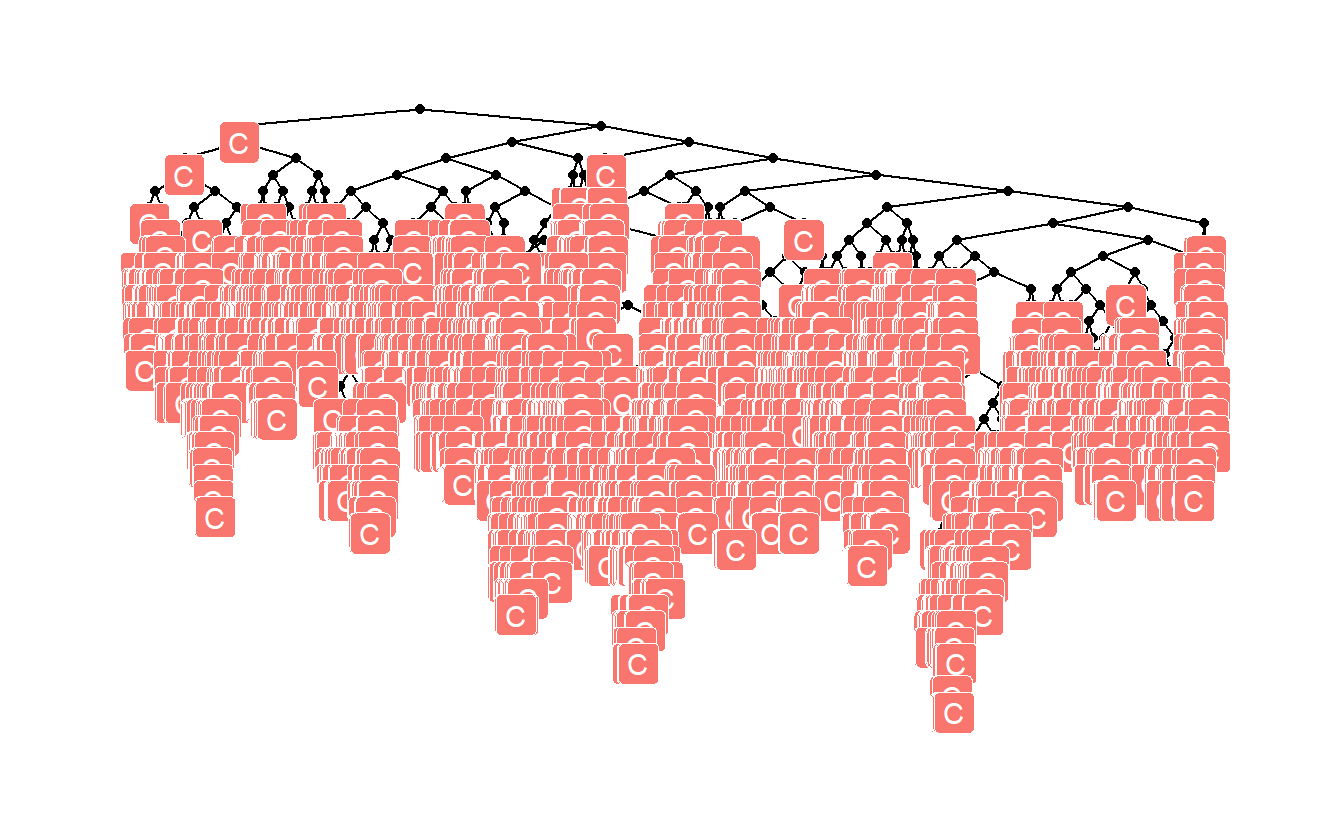Random Forest models normally come equipped with feature importances:

varImpPlot(rf_model)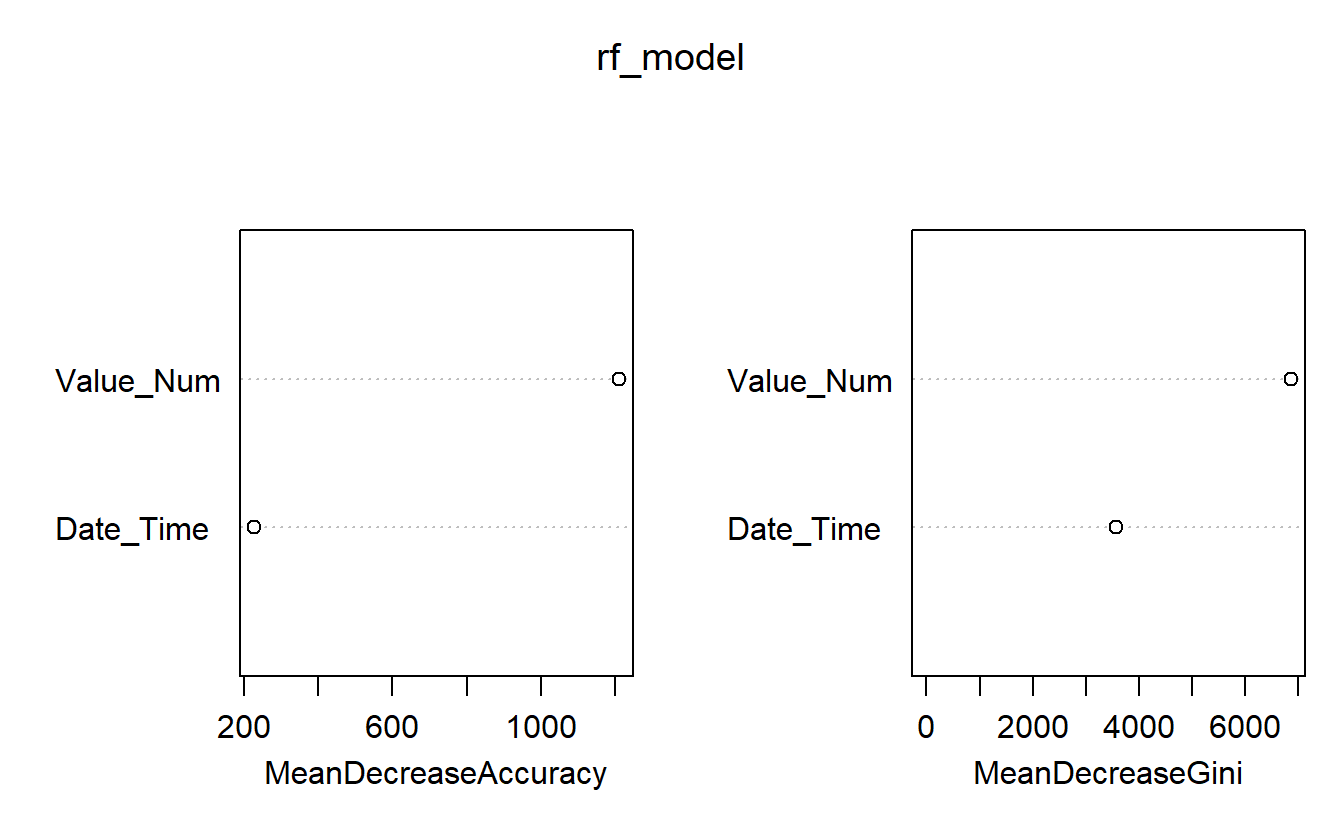Feature Importances of Base Model

For instance, above we see that Date_Time is more in terms classifying the measurement as opposed to Value_Num

We can also extract the feature importances for each of the classes:

caret::varImp(rf_model, scale = FALSE) %>%
t() %>%  # transpose the entire dataframe
as_tibble(rownames = 'Class' ) # turn it back to a tibble
#> # A tibble: 17 x 3
#>   Class Date_Time Value_Num
#>   <chr>     <dbl>     <dbl>
#> 1 C_33   0.0969     0.517
#> 2 C_34   0.264      0.509
#> 3 C_35   0.373      0.507
#> 4 C_58   0.0481     0.246
#> 5 C_59  -0.000723  -0.00106
#> 6 C_60   0.0413     0.194
#> # ... with 11 more rows

The Random Forest model also has a plot associated with it:

plot(rf_model)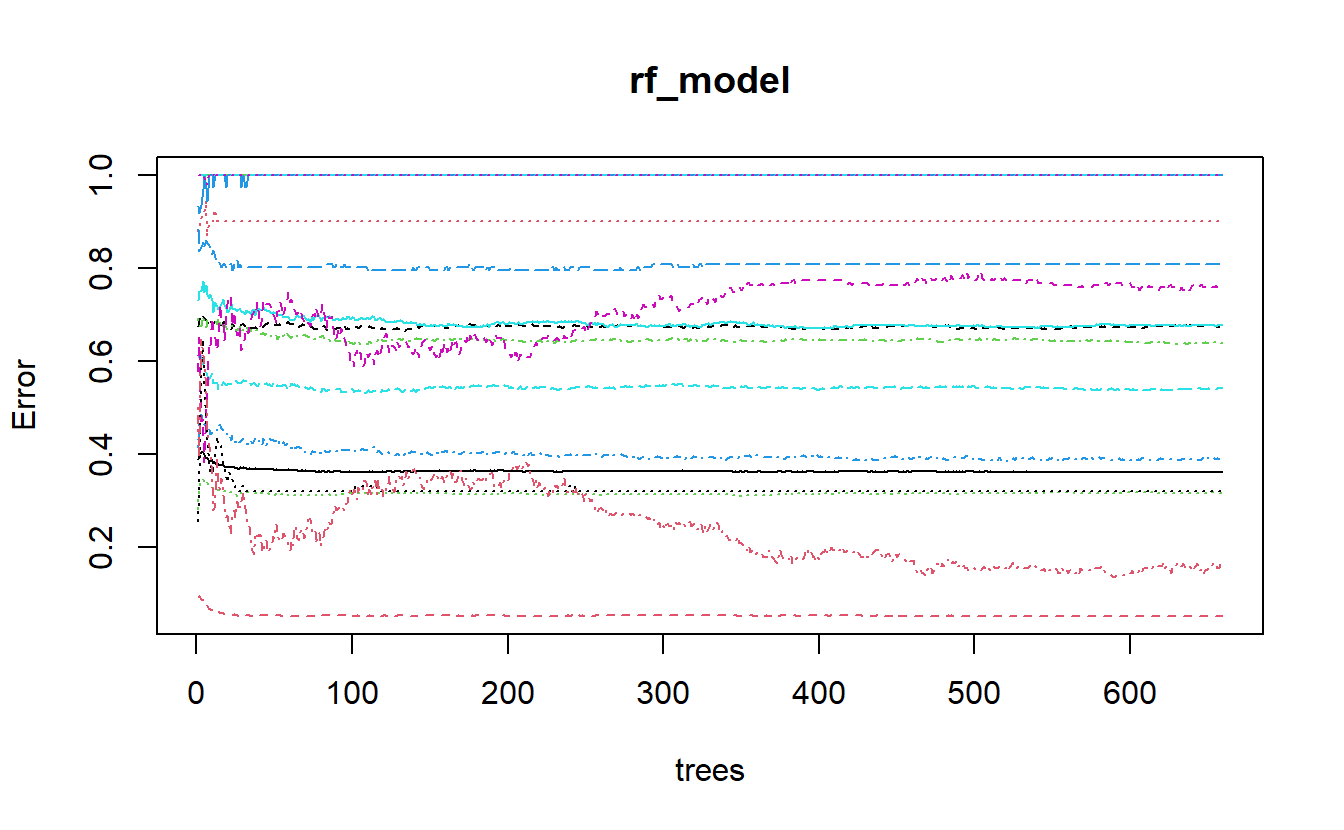plot of randomForest model output

Let’s see if we can figure out what is going on with the above graph. We can extract the err.rate and ntree from the model object and place them in a matplot to match the above graph.

err <- rf_model$err.rate N_trees <- 1:rf_model$ntree

matplot(N_trees , err, type = 'l', xlab="trees", ylab="Error")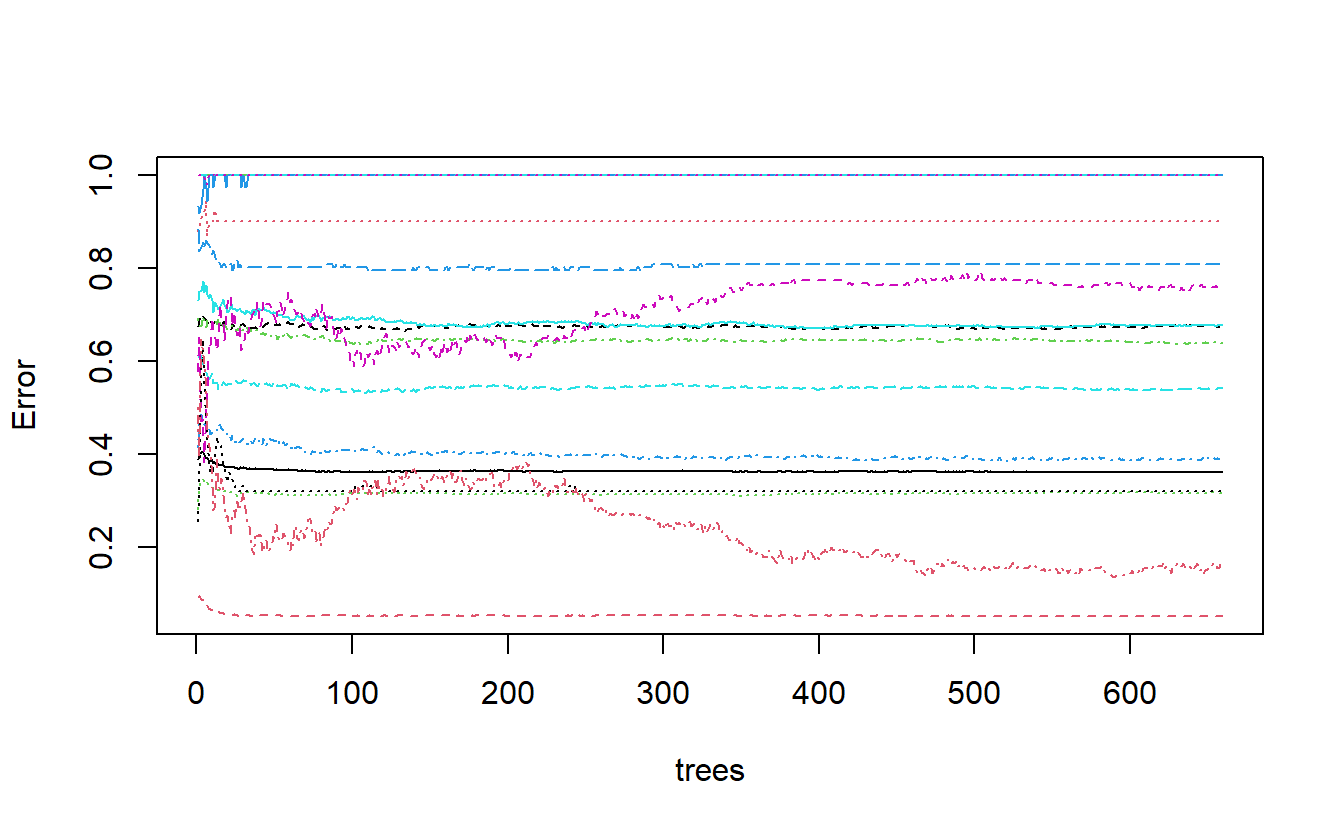derivation of plot of randomForest model output

We can improve this a bit by performing the computations ourselves and keeping track of things:

err2 <- as_tibble(err, rownames='n_trees')

err2 %>%
glimpse()
#> Rows: 659
#> Columns: 19
#> $n_trees <chr> "1", "2", "3", "4", "5", "6", "7", "8", "9", "10", "11", "12",~ #>$ OOB     <dbl> 0.3888985, 0.4010195, 0.4031778, 0.4038520, 0.3991778, 0.39185~
#> $C_33 <dbl> 0.09125475, 0.09435626, 0.08716876, 0.08636837, 0.08526068, 0.~ #>$ C_34    <dbl> 0.2818713, 0.3272194, 0.3405500, 0.3448990, 0.3397964, 0.33502~
#> $C_35 <dbl> 0.4140625, 0.4623116, 0.4863732, 0.4646840, 0.4636678, 0.45928~ #>$ C_58    <dbl> 0.6084724, 0.6100235, 0.6091371, 0.5932394, 0.5891270, 0.58295~
#> $C_59 <dbl> 1, 1, 1, 1, 1, 1, 1, 1, 1, 1, 1, 1, 1, 1, 1, 1, 1, 1, 1, 1, 1,~ #>$ C_60    <dbl> 0.6745363, 0.6883768, 0.7014563, 0.6958763, 0.6922034, 0.68802~
#> $C_61 <dbl> 0.8823529, 0.8695652, 0.9062500, 0.9166667, 0.9166667, 0.94444~ #>$ C_62    <dbl> 0.6921986, 0.6770186, 0.6747851, 0.6957352, 0.6849882, 0.67257~
#> $C_63 <dbl> 0.8837209, 0.8354430, 0.8446602, 0.8547009, 0.8412698, 0.85937~ #>$ C_64    <dbl> 0.7313433, 0.7507692, 0.7500000, 0.7707424, 0.7479508, 0.76247~
#> $C_65 <dbl> 0.5774648, 0.6504065, 0.4697987, 0.4756098, 0.3815029, 0.47777~ #>$ C_66    <dbl> 0.2564103, 0.3000000, 0.5769231, 0.6470588, 0.5617978, 0.48351~
#> $C_67 <dbl> 0.5000000, 0.3931624, 0.6466667, 0.6190476, 0.6032609, 0.53968~ #>$ C_68    <dbl> 1, 1, 1, 1, 1, 1, 1, 1, 1, 1, 1, 1, 1, 1, 1, 1, 1, 1, 1, 1, 1,~
#> $C_69 <dbl> 0.9333333, 0.9166667, 0.9354839, 0.9696970, 1.0000000, 0.97142~ #>$ C_70    <dbl> 1, 1, 1, 1, 1, 1, 1, 1, 1, 1, 1, 1, 1, 1, 1, 1, 1, 1, 1, 1, 1,~
#> $C_71 <dbl> 1.0000000, 1.0000000, 1.0000000, 1.0000000, 1.0000000, 0.98076~ predicted_levels <- colnames(err2)[!colnames(err2) %in% c('n_trees','OOB')] predicted_levels #>  "C_33" "C_34" "C_35" "C_58" "C_59" "C_60" "C_61" "C_62" "C_63" "C_64" #>  "C_65" "C_66" "C_67" "C_68" "C_69" "C_70" "C_71" plot <- err2 %>% select(-OOB) %>% pivot_longer(all_of(predicted_levels), names_to = "Class", values_to = "Error") %>% mutate(N_Trees = as.numeric(n_trees)) %>% ggplot(aes(x=N_Trees, y=Error, color=Class)) + geom_point() + geom_smooth(method = lm, formula = y ~ splines::bs(x, 3), se = FALSE) plot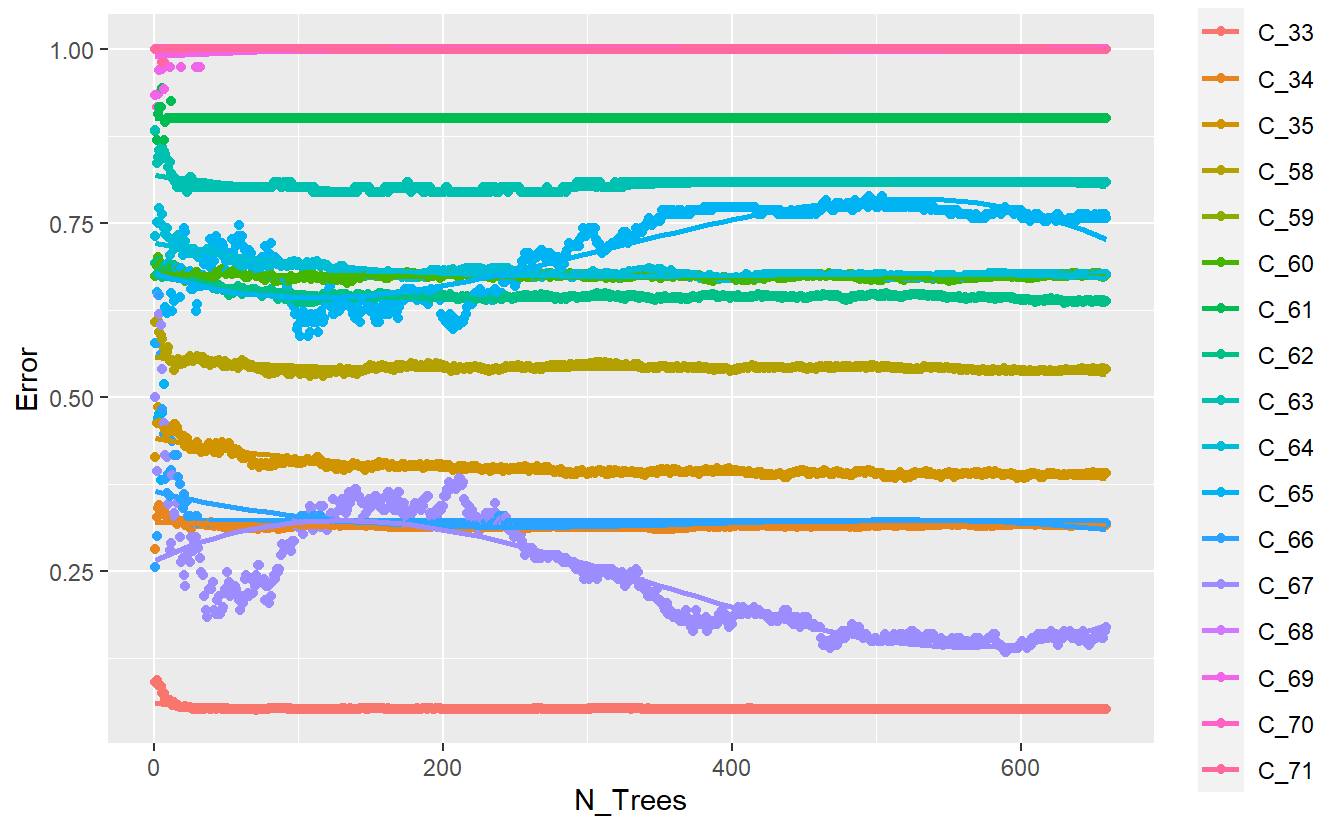Improved plot of base randomForest model output better_rand_forest_errors <- function(rf_model_obj){ err <- rf_model_obj$err.rate

err2 <- as_tibble(err, rownames='n_trees')

predicted_levels <- colnames(err2)[!colnames(err2) %in% c('n_trees','OOB')]

plot <- err2 %>%
select(-OOB) %>%
pivot_longer(all_of(predicted_levels), names_to = "Class", values_to = "Error") %>%
mutate(N_Trees = as.numeric(n_trees)) %>%
ggplot(aes(x=N_Trees, y=Error, color=Class)) +
geom_point() +
geom_smooth(method = lm, formula = y ~ splines::bs(x, 3), se = FALSE)

return(plot)
}

$$~$$

$$~$$

## 13.8 Evaluate Model Performace

DATA.test.Scored <- DATA.test %>%
mutate(model = 'base')

First, we will get the expected classes for the test data:

DATA.test.Scored$pred <- predict(rf_model, DATA.test , 'response') DATA.test.Scored %>% glimpse() #> Rows: 10,464 #> Columns: 15 #>$ Date             <chr> "04-21-1991", "04-21-1991", "04-22-1991", "04-22-1991~
#> $Time <chr> "9:09", "9:09", "7:35", "7:35", "7:25", "7:29", "7:29~ #>$ Code             <dbl> 58, 34, 58, 34, 58, 58, 33, 34, 33, 62, 33, 62, 33, 3~
#> $Value <chr> "100", "013", "216", "013", "257", "067", "009", "014~ #>$ file_no          <dbl> 1, 1, 1, 1, 1, 1, 1, 1, 1, 1, 1, 1, 1, 1, 1, 1, 1, 1,~
#> $Date_Time_Char <chr> "04-21-1991 9:09", "04-21-1991 9:09", "04-22-1991 7:3~ #>$ Date_Time        <dttm> 1991-04-21 09:09:00, 1991-04-21 09:09:00, 1991-04-22~
#> $Value_Num <dbl> 100, 13, 216, 13, 257, 67, 9, 14, 2, 228, 7, 256, 8, ~ #>$ Record_ID        <int> 1, 3, 7, 9, 13, 25, 26, 27, 32, 37, 38, 42, 43, 45, 5~
#> $Code_Description <chr> "Pre-breakfast blood glucose measurement", "NPH insul~ #>$ Code_Invalid     <lgl> FALSE, FALSE, FALSE, FALSE, FALSE, FALSE, FALSE, FALS~
#> $Code_New <dbl> 58, 34, 58, 34, 58, 58, 33, 34, 33, 62, 33, 62, 33, 3~ #>$ Code_Factor      <fct> C_58, C_34, C_58, C_34, C_58, C_58, C_33, C_34, C_33,~
#> $model <chr> "base", "base", "base", "base", "base", "base", "base~ #>$ pred             <fct> C_60, C_35, C_58, C_35, C_62, C_63, C_33, C_33, C_33,~
library('yardstick')
#> For binary classification, the first factor level is assumed to be the event.
#> Use the argument event_level = "second" to alter this as needed.
#>
#> Attaching package: 'yardstick'
#>
#>     spec
conf_mat.DATA.test.Scored <- conf_mat(DATA.test.Scored,
truth = Code_Factor, pred)
conf_mat.DATA.test.Scored %>%
autoplot('mosaic')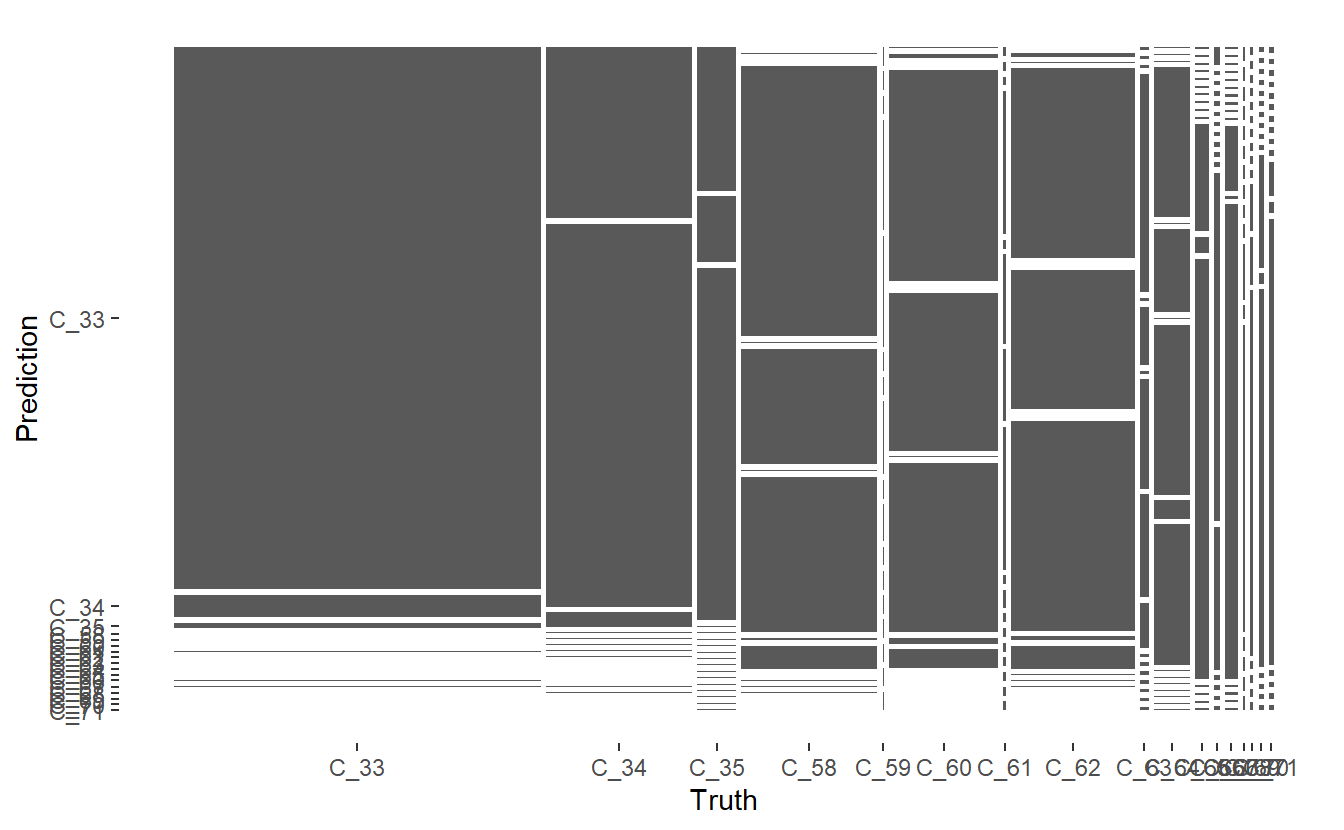Mosaic Confusion Matrix of Base Model

DATA.test.Scored %>%
conf_mat(truth= Code_Factor, pred) %>%
summary() %>%
ggplot(aes(x=.metric, y=.estimate, fill= .metric)) +
geom_bar(stat='identity', position = 'dodge') +
coord_flip()
#> Warning: While computing multiclass precision(), some levels had no predicted events (i.e. true_positive + false_positive = 0).
#> Precision is undefined in this case, and those levels will be removed from the averaged result.
#> Note that the following number of true events actually occured for each problematic event level:
#> 'C_68': 13
#> 'C_69': 29
#> 'C_70': 49
#> 'C_71': 43

#> Warning: While computing multiclass precision(), some levels had no predicted events (i.e. true_positive + false_positive = 0).
#> Precision is undefined in this case, and those levels will be removed from the averaged result.
#> Note that the following number of true events actually occured for each problematic event level:
#> 'C_68': 13
#> 'C_69': 29
#> 'C_70': 49
#> 'C_71': 43
#> Warning: Removed 1 rows containing missing values (geom_bar).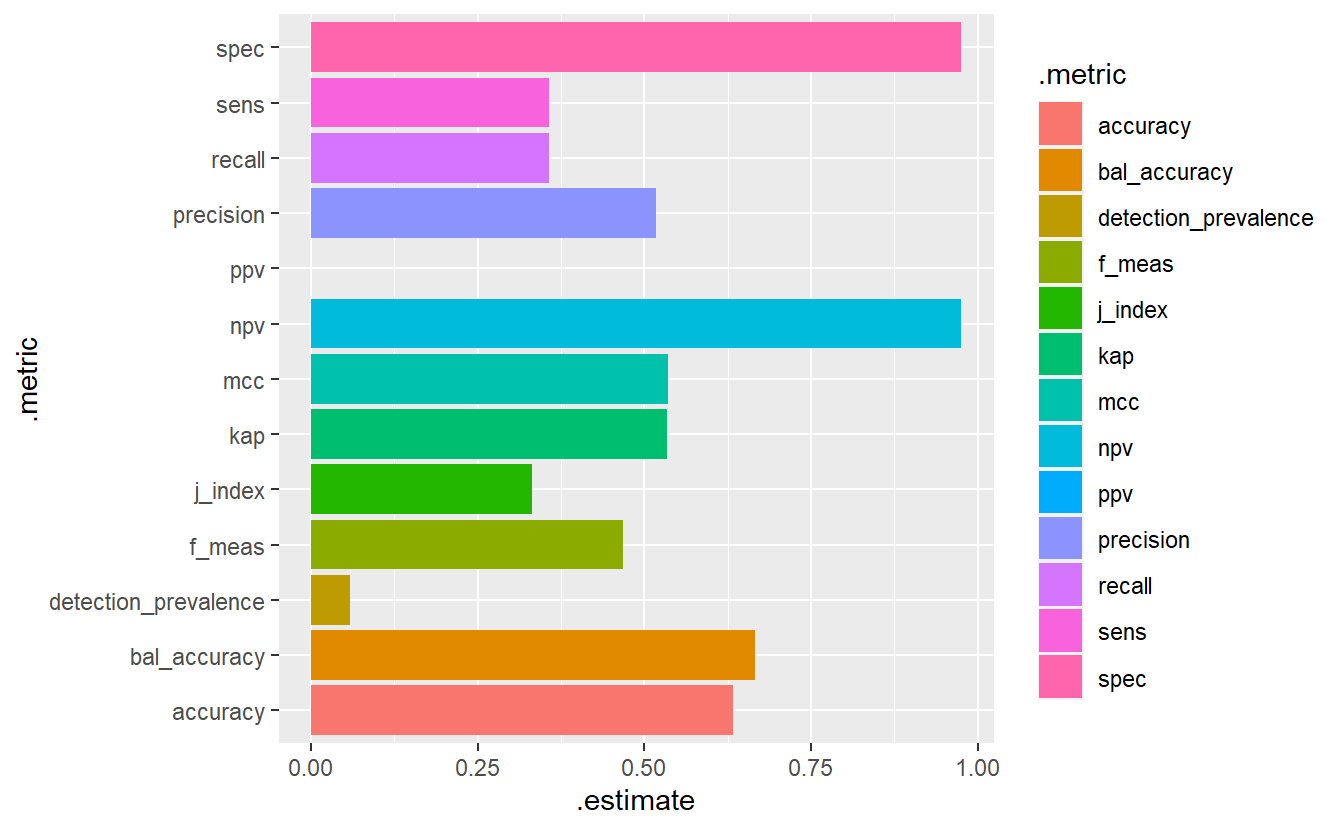Model Evaluation Metrics - Base Model

DATA.test.Scored %>%
mutate(Predict_Correct = if_else(Code_Factor == pred, TRUE, FALSE)) %>%
ggplot(aes(x=Date_Time, y=Value_Num, color=Predict_Correct)) +
geom_point()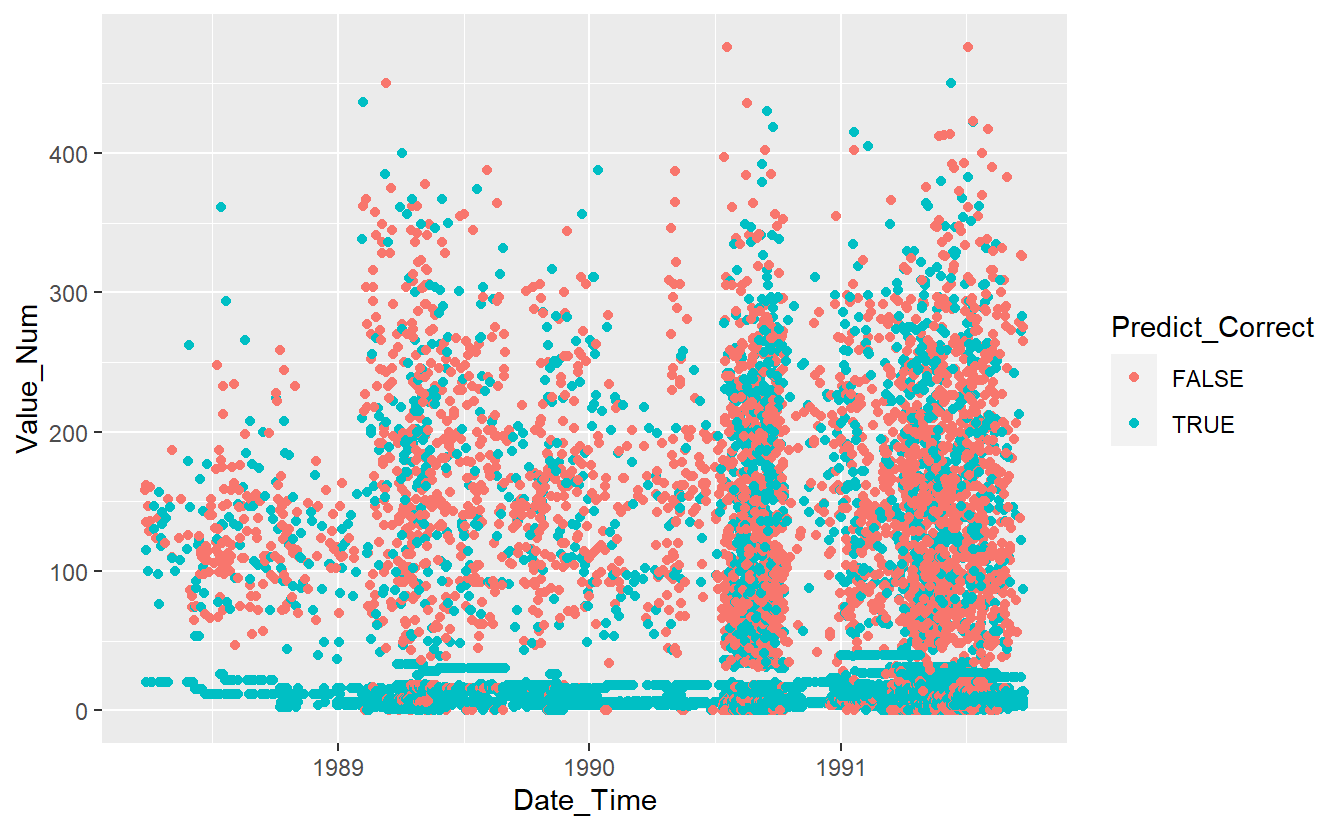Graph of Correct Predictions on test data under base model

$$~$$

$$~$$

## 13.9 Correlated Probabilites

First, let’s review the probabilities:

Probs <- predict(rf_model, DATA.test.Scored, 'prob') %>%
as_tibble()

Probs
#> # A tibble: 10,464 x 17
#>   C_33   C_34  C_35  C_58  C_59  C_60  C_61  C_62  C_63  C_64  C_65  C_66  C_67
#>   <matr> <mat> <mat> <mat> <mat> <mat> <mat> <mat> <mat> <mat> <mat> <mat> <mat>
#> 1 0.000~ 0.00~ 0.00~ 0.02~ 0     0.55~ 0     0.37~ 0.00~ 0.03~ 0     0     0
#> 2 0.250~ 0.00~ 0.74~ 0.00~ 0     0.00~ 0     0.00~ 0.00~ 0.00~ 0     0     0
#> 3 0.000~ 0.00~ 0.00~ 0.69~ 0     0.16~ 0     0.13~ 0.00~ 0.00~ 0     0     0
#> 4 0.251~ 0.01~ 0.73~ 0.00~ 0     0.00~ 0     0.00~ 0.00~ 0.00~ 0     0     0
#> 5 0.000~ 0.00~ 0.00~ 0.31~ 0     0.20~ 0     0.42~ 0.00~ 0.05~ 0     0     0
#> 6 0.000~ 0.00~ 0.00~ 0.15~ 0     0.28~ 0     0.21~ 0.28~ 0.05~ 0     0     0
#> # ... with 10,458 more rows, and 4 more variables: C_68 <matrix>,
#> #   C_69 <matrix>, C_70 <matrix>, C_71 <matrix>

DATA.test.Scored <- cbind(DATA.test.Scored, Probs)
GGally::ggcorr(Probs,
method = c("pairwise", "pearson"),
label = TRUE)
#> Registered S3 method overwritten by 'GGally':
#>   method from
#>   +.gg   ggplot2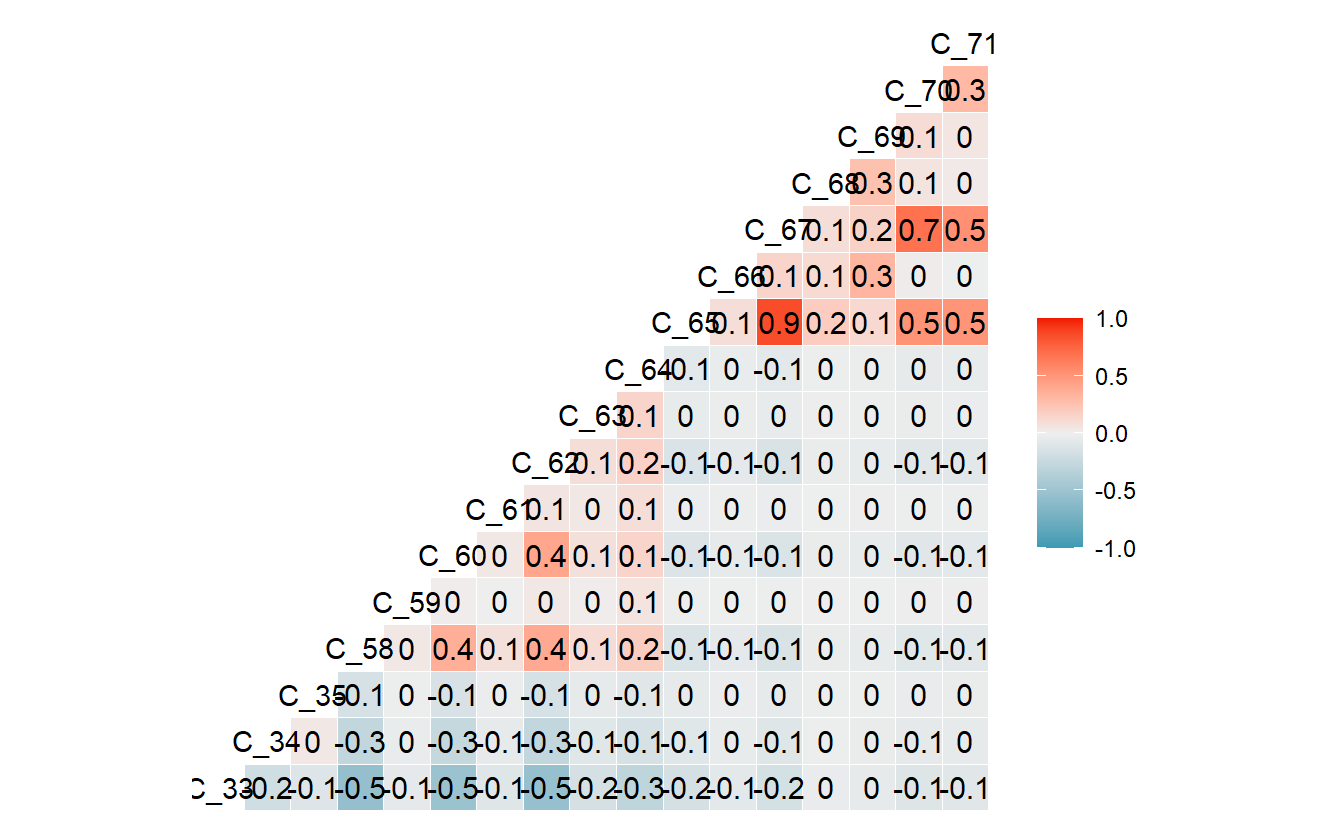library('corrr')
cor.corr_data <- correlate(Probs)
#>
#> Correlation method: 'pearson'
#> Missing treated using: 'pairwise.complete.obs'

cor.corr_data %>%
glimpse()
#> Rows: 17
#> Columns: 18
#> $term <chr> "C_33", "C_34", "C_35", "C_58", "C_59", "C_60", "C_61", "C_62", "~ #>$ C_33 <dbl> NA, -0.19163898, -0.10441614, -0.54956761, -0.05396652, -0.511840~
#> $C_34 <dbl> -0.19163898, NA, 0.04132957, -0.28371748, -0.02844334, -0.2606538~ #>$ C_35 <dbl> -0.10441614, 0.04132957, NA, -0.14865214, -0.01483954, -0.1393353~
#> $C_58 <dbl> -0.54956761, -0.28371748, -0.14865214, NA, 0.03561936, 0.36949568~ #>$ C_59 <dbl> -0.053966522, -0.028443345, -0.014839537, 0.035619361, NA, 0.0124~
#> $C_60 <dbl> -0.51184049, -0.26065388, -0.13933531, 0.36949568, 0.01240024, NA~ #>$ C_61 <dbl> -0.097743416, -0.050999090, -0.023185268, 0.081489225, -0.0013966~
#> $C_62 <dbl> -0.54217945, -0.27453263, -0.14798142, 0.39238423, 0.03940021, 0.~ #>$ C_63 <dbl> -0.164511288, -0.086079561, -0.045169014, 0.105786617, 0.01077281~
#> $C_64 <dbl> -0.29676777, -0.14955872, -0.08073562, 0.18846421, 0.05790872, 0.~ #>$ C_65 <dbl> -0.16073437, -0.08587324, -0.04520394, -0.12428883, -0.01218820, ~
#> $C_66 <dbl> -0.066317537, -0.038630694, -0.020294164, -0.056104543, -0.005503~ #>$ C_67 <dbl> -0.16848325, -0.09130119, -0.04765189, -0.13115047, -0.01286117, ~
#> $C_68 <dbl> -0.029782995, -0.015318848, -0.010093123, -0.027725505, -0.002723~ #>$ C_69 <dbl> -0.042576706, -0.026037345, -0.013980500, -0.038633843, -0.003788~
#> $C_70 <dbl> -0.108371422, -0.059014542, -0.030955034, -0.085714612, -0.008407~ #>$ C_71 <dbl> -0.083580597, -0.048190858, -0.026590249, -0.073846899, -0.007241~
conf_mat.DATA.test.Scored %>%
autoplot('heatmap')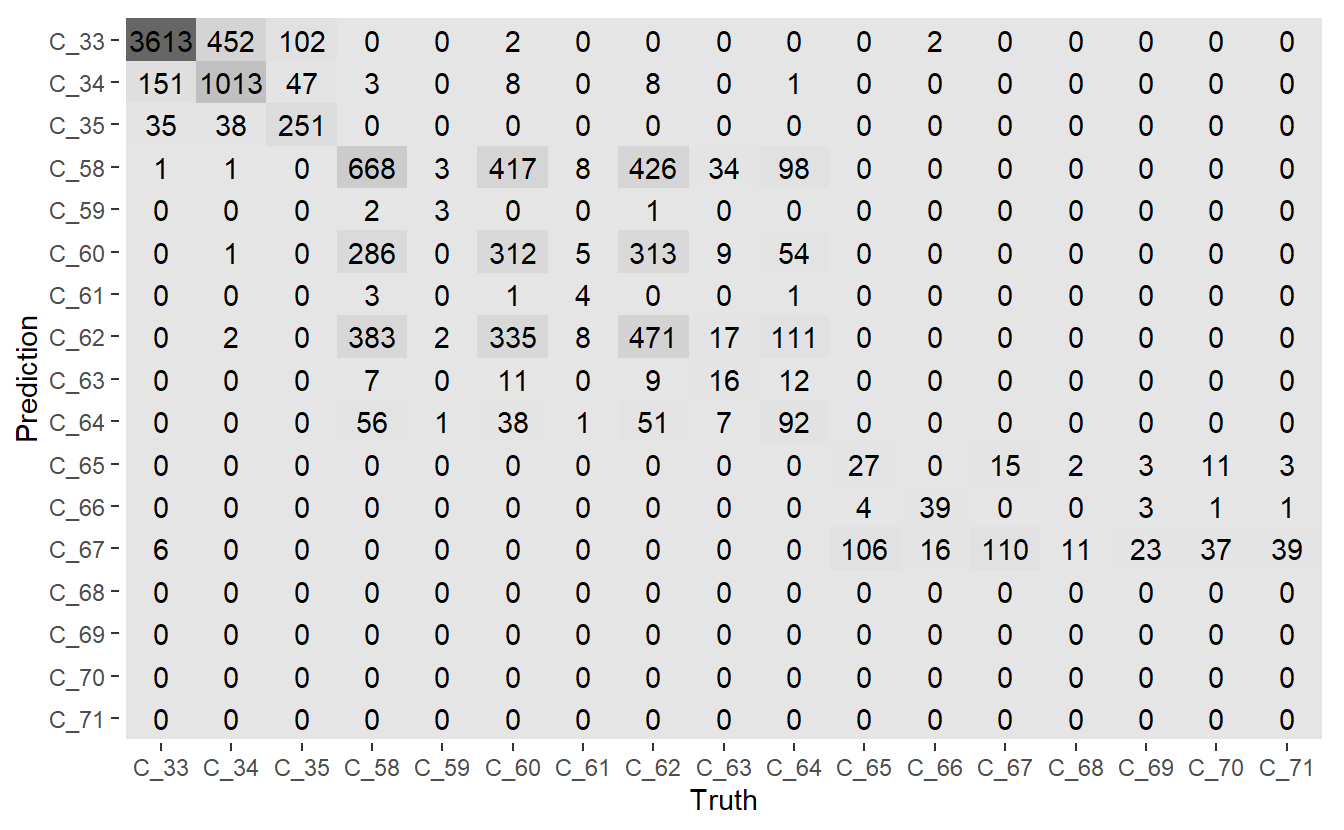Heatmap Confusion Matrix of base model

One of the things to note above is that classes C_68, C_69, C_70, C_71 are never predicted.

Likewise the probabilities of C_65, C_70, and C_67 are highly correlated:

cor.corr_data %>%
filter_at(vars(all_of(colnames(Probs))), any_vars( . > .6 ))
#> # A tibble: 3 x 18
#>   term    C_33    C_34    C_35    C_58     C_59    C_60    C_61    C_62    C_63
#>   <chr>  <dbl>   <dbl>   <dbl>   <dbl>    <dbl>   <dbl>   <dbl>   <dbl>   <dbl>
#> 1 C_65  -0.161 -0.0859 -0.0452 -0.124  -0.0122  -0.116  -0.0221 -0.123  -0.0372
#> 2 C_67  -0.168 -0.0913 -0.0477 -0.131  -0.0129  -0.122  -0.0233 -0.129  -0.0392
#> 3 C_70  -0.108 -0.0590 -0.0310 -0.0857 -0.00841 -0.0798 -0.0152 -0.0846 -0.0256
#> # ... with 8 more variables: C_64 <dbl>, C_65 <dbl>, C_66 <dbl>, C_67 <dbl>,
#> #   C_68 <dbl>, C_69 <dbl>, C_70 <dbl>, C_71 <dbl>

And there are a number of classes which do not have many training examples:

DATA.train %>%
group_by(Code_Factor) %>%
tally() %>%
ungroup() %>%
mutate(n = as.numeric(n)) %>%
full_join(Code_Table_Factor) %>%
mutate(n = if_else(is.na(n) , 0 , n)) %>%
arrange(n) %>%
print(n=20)
#> Joining, by = "Code_Factor"
#> # A tibble: 17 x 6
#>    Code_Factor     n  Code Code_Description                Code_Invalid Code_New
#>    <fct>       <dbl> <dbl> <chr>                           <lgl>           <dbl>
#>  1 C_59           11    59 Post-breakfast blood glucose m~ FALSE              59
#>  2 C_68           21    68 Less-than-usual meal ingestion  FALSE              68
#>  3 C_69           39    69 Typical exercise activity       FALSE              69
#>  4 C_61           40    61 Post-lunch blood glucose measu~ FALSE              61
#>  5 C_71           55    71 Less-than-usual exercise activ~ FALSE              71
#>  6 C_70           90    70 More-than-usual exercise activ~ FALSE              70
#>  7 C_66           97    66 Typical meal ingestion          FALSE              66
#>  8 C_63          136    63 Post-supper blood glucose meas~ FALSE              63
#>  9 C_65          194    65 Hypoglycemic symptoms           FALSE              65
#> 10 C_67          201    67 More-than-usual meal ingestion  FALSE              67
#> 11 C_64          535    64 Pre-snack blood glucose measur~ FALSE              64
#> 12 C_35          653    35 UltraLente insulin dose         FALSE              35
#> 13 C_60         1643    60 Pre-lunch blood glucose measur~ FALSE              60
#> 14 C_62         1877    62 Pre-supper blood glucose measu~ FALSE              62
#> 15 C_58         2108    58 Pre-breakfast blood glucose me~ FALSE              58
#> 16 C_34         2321    34 NPH insulin dose                FALSE              34
#> 17 C_33         5674    33 Regular insulin dose            FALSE              33
Probs.sample <- Probs %>%
rowwise() %>%
mutate(rand_num = runif(1)) %>%
ungroup() %>%
filter(rand_num > .4) %>%
select(-rand_num) # this should sample roughly 60% of the Probs data

nrow(Probs.sample)/nrow(Probs)
#>  0.5952791
library('broom')
proc.kmeans <- function(data, k = 1:9){

kclusts <-
tibble(k = k) %>%
mutate(
kclust = map(k, ~kmeans(data, .x)),
glanced = map(kclust, glance),
augmented = map(kclust, augment, data)
)

kmeans_model <- function(final_k = 5){
(kclusts %>%
filter(k == final_k))$kclust[] } id_vars <- colnames(kclusts) cluster_assignments <- kclusts %>% unnest(cols = augmented) vars <- setdiff(colnames(cluster_assignments), c(id_vars,'.cluster')) within_cluster_variation_plot <- kclusts %>% unnest(cols = c(glanced)) %>% ggplot(aes(k, tot.withinss)) + geom_line() + geom_point() + labs(title = "Within Cluster Variation versus number of Clusters") kmeans.pca <- cluster_assignments %>% select(all_of(vars)) %>% prcomp() cluster_assignments_plot <- kmeans.pca %>% augment(cluster_assignments) %>% ggplot( aes(x = .fittedPC1, y = .fittedPC2, color = .cluster )) + geom_point(alpha = 0.8) + facet_wrap(~ k) + labs(title = "k-means clusterings") return(list(cluster_assignments_plot= cluster_assignments_plot, within_cluster_variation_plot=within_cluster_variation_plot, kmeans_model = kmeans_model)) } proc.kmeans.results <- proc.kmeans(Probs.sample, k=3:11) proc.kmeans.results #>$cluster_assignments_plot
#>
#> $within_cluster_variation_plot #> #>$kmeans_model
#> function(final_k = 5){
#>    (kclusts %>%
#>      filter(k == final_k))$kclust[] #> } #> <environment: 0x000000001d5d4230>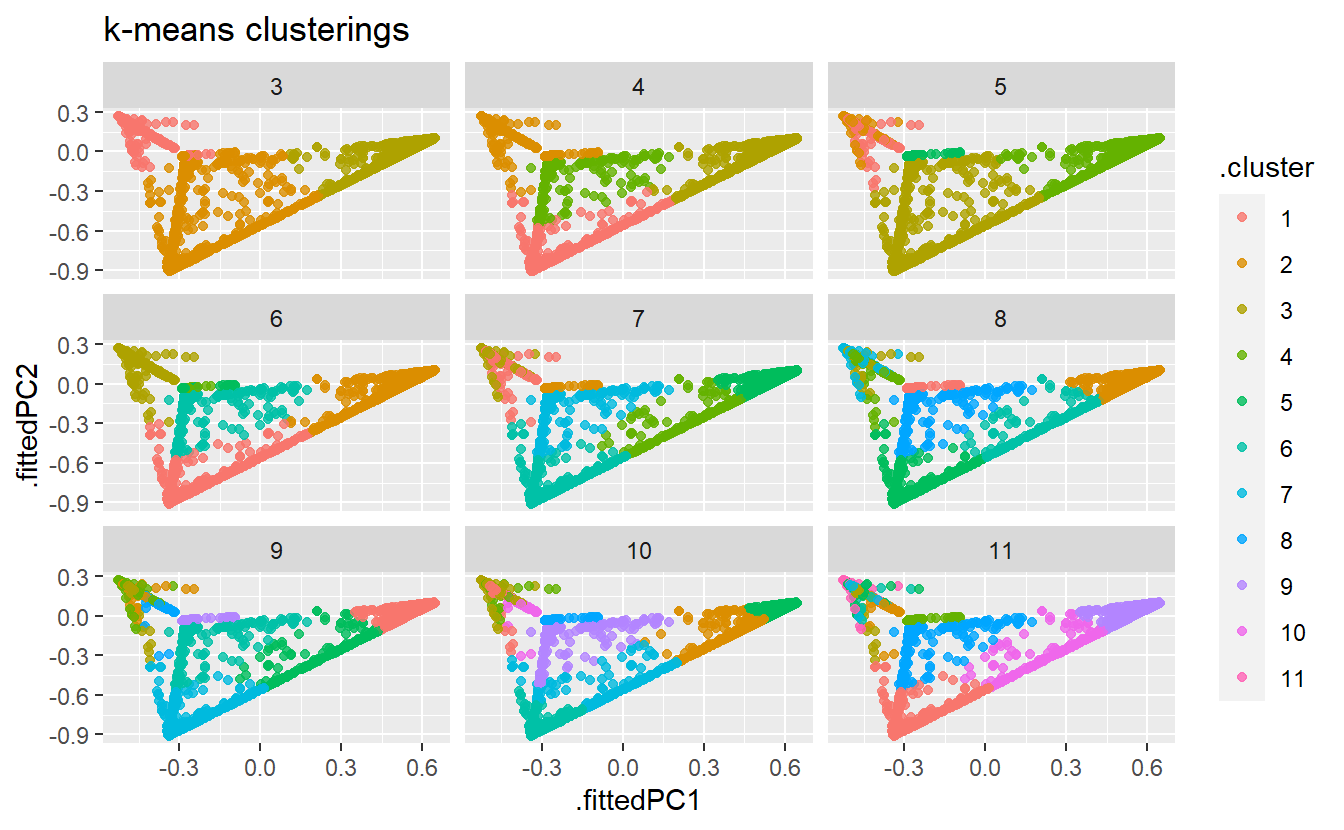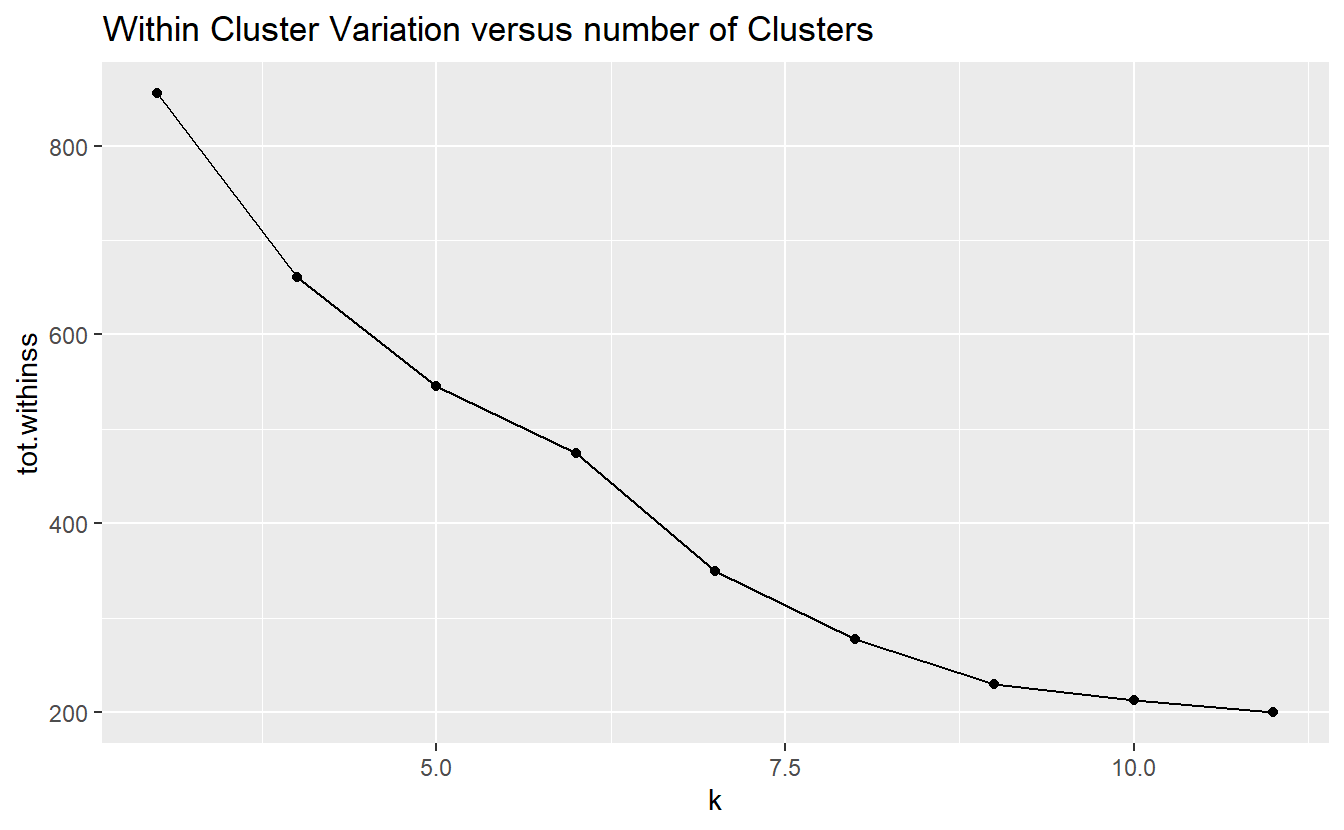proc.kmeans.results$kmeans_model(6) %>%
augment(Probs.sample) %>%
rownames_to_column("id") %>%
pivot_longer(cols = c(-.cluster, -id), names_to = "class", values_to = "prob") %>%
mutate(prob = as.numeric(prob)) %>%
arrange(desc(prob)) %>%
ggplot(aes(x=class, y=prob, color = .cluster)) +
geom_point() +
facet_wrap(~.cluster)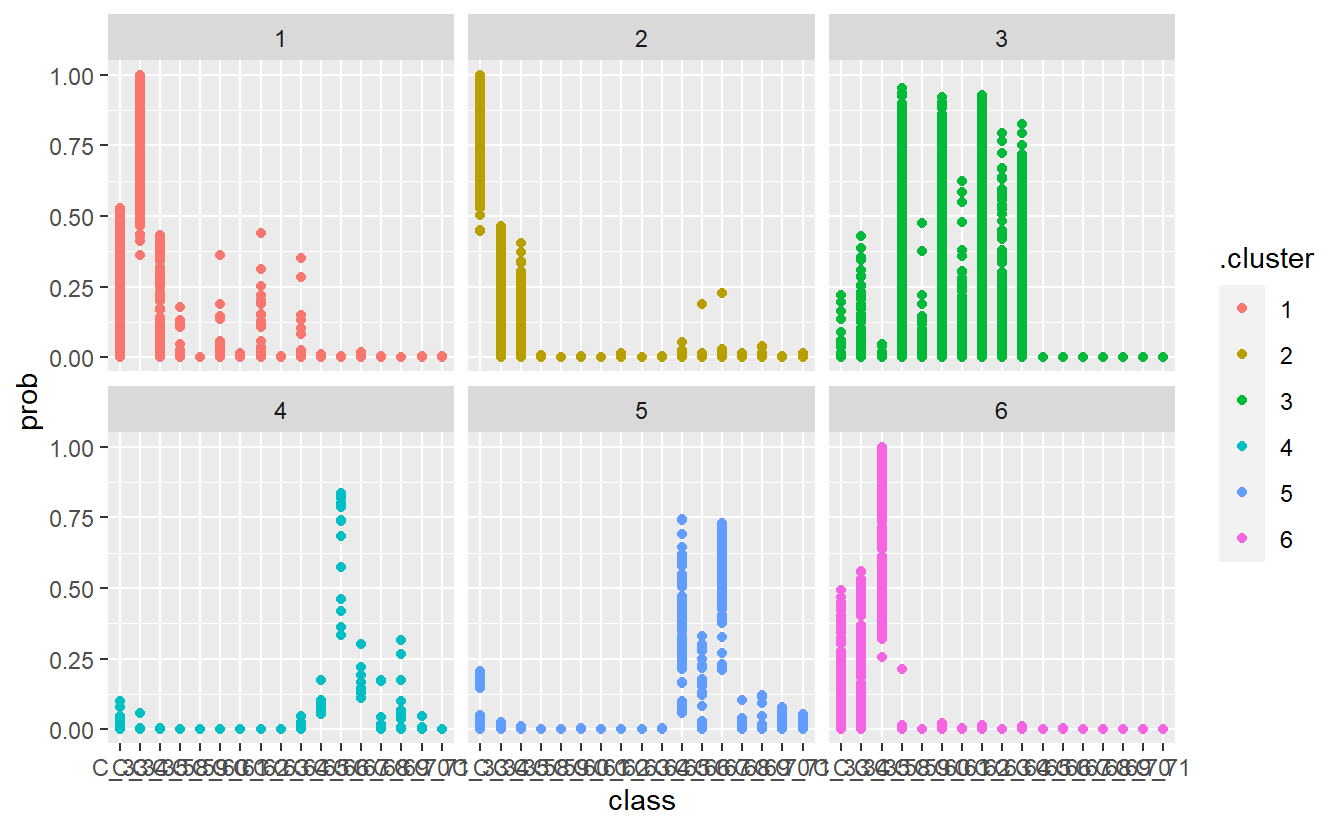proc.kmeans.results$kmeans_model(6) %>% augment(Probs.sample) %>% rownames_to_column("id") %>% pivot_longer(cols = c(-.cluster, -id), names_to = "class", values_to = "prob") %>% mutate(prob = as.numeric(prob)) %>% arrange(desc(prob)) %>% filter(.cluster == 3) %>% ggplot(aes(x=class, y=prob, color = .cluster)) + geom_point()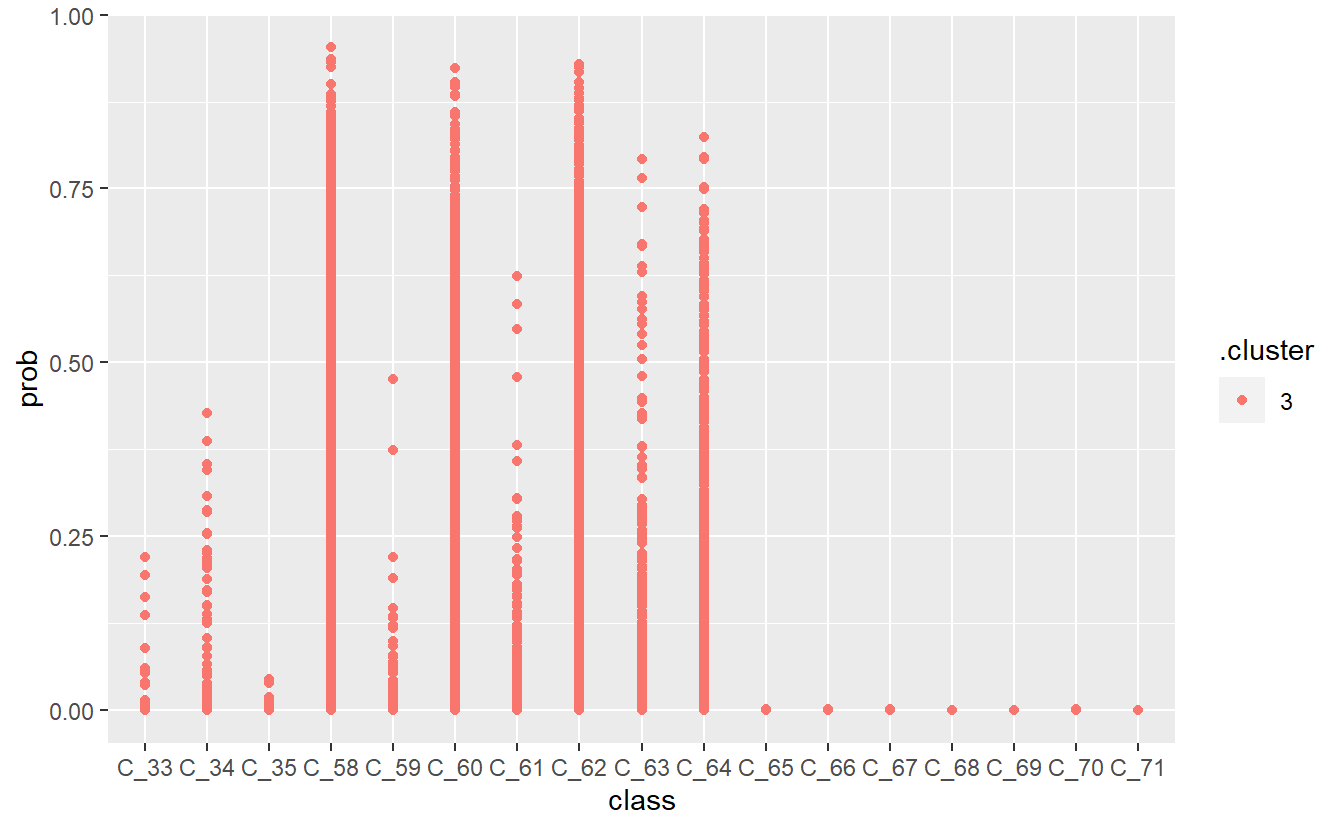rm(proc.kmeans.results) saveRDS(rf_model,"DATA/Part_5/models/rf_model.RDS") rm(rf_model) ## 13.10 Make Adjustments Our previous model has some issues, which we can attempt to correct. Code_Table_Factor_2 <- Code_Table_Factor %>% mutate(Code_Description_2 = case_when( Code %in% c(33, 34, 35) ~ "insulin dose", Code %in% c(58, 60, 62, 64) ~ "pre-meal", Code %in% c(59, 61, 63) ~ "post-meal", Code %in% c(66, 67, 68, 65) ~ "meal ingestion / Hypoglycemic", Code %in% c(69, 70, 71) ~ "exercise activity") ) %>% mutate(Code_Description_2 = factor(Code_Description_2)) %>% select(Code, Code_Description_2) Code_Table_Factor_2 #> # A tibble: 17 x 2 #> Code Code_Description_2 #> <dbl> <fct> #> 1 33 insulin dose #> 2 34 insulin dose #> 3 35 insulin dose #> 4 58 pre-meal #> 5 59 post-meal #> 6 60 pre-meal #> # ... with 11 more rows DATA.train_2 <- DATA.train %>% left_join(Code_Table_Factor_2) #> Joining, by = "Code" rf_model_2 <- randomForest(Code_Description_2 ~ Date_Time + Value_Num, data = DATA.train_2, ntree = 659, importance = TRUE) better_rand_forest_errors(rf_model_2)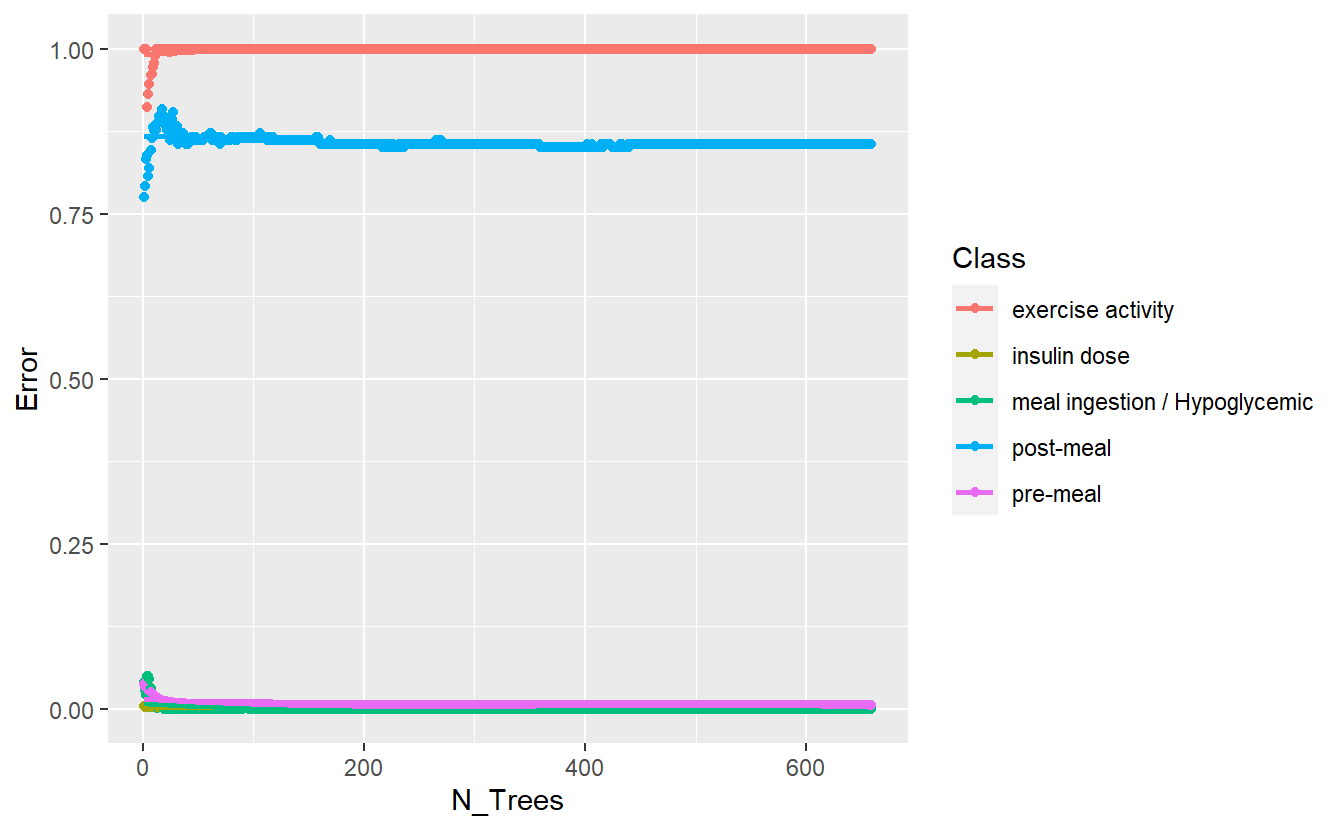Training Errors on New Model DATA.test.Scored2 <- DATA.test %>% left_join(Code_Table_Factor_2) %>% mutate(model = 'new') #> Joining, by = "Code" DATA.test.Scored2$pred <- predict(rf_model_2, DATA.test.Scored2 , 'response')

conf_mat.DATA.test.Scored2 <- DATA.test.Scored2 %>%
conf_mat(truth = Code_Description_2, pred)

conf_mat.DATA.test.Scored2 %>%
autoplot('heatmap')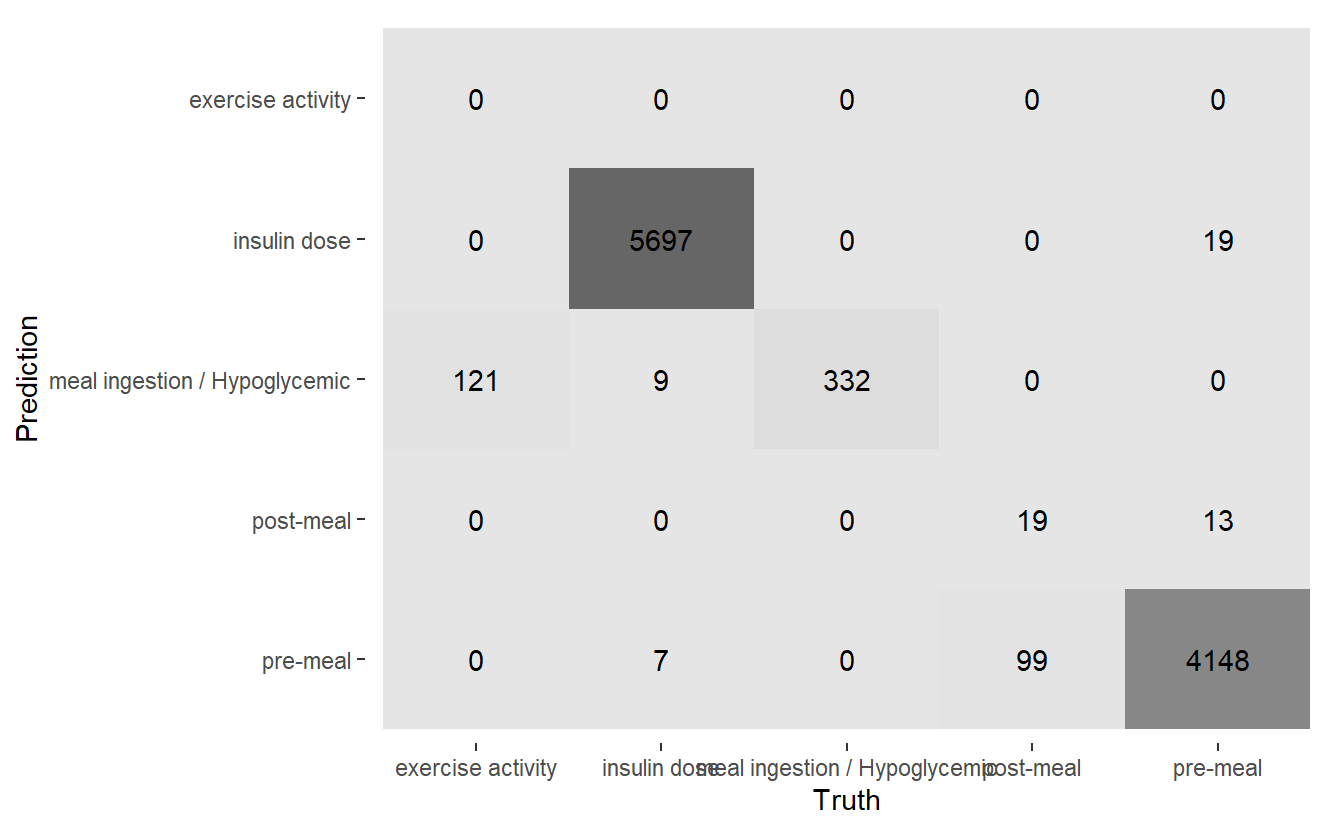Heatmap Confusion Matrix of New Model

saveRDS(rf_model_2, 'DATA/Part_5/models/rf_model_2.RDS')
base <- DATA.test.Scored %>% conf_mat(truth= Code_Factor, pred) %>% summary() %>% mutate(model = 'base')
#> Warning: While computing multiclass precision(), some levels had no predicted events (i.e. true_positive + false_positive = 0).
#> Precision is undefined in this case, and those levels will be removed from the averaged result.
#> Note that the following number of true events actually occured for each problematic event level:
#> 'C_68': 13
#> 'C_69': 29
#> 'C_70': 49
#> 'C_71': 43

#> Warning: While computing multiclass precision(), some levels had no predicted events (i.e. true_positive + false_positive = 0).
#> Precision is undefined in this case, and those levels will be removed from the averaged result.
#> Note that the following number of true events actually occured for each problematic event level:
#> 'C_68': 13
#> 'C_69': 29
#> 'C_70': 49
#> 'C_71': 43
new <- DATA.test.Scored2 %>% conf_mat(truth= Code_Description_2, pred) %>% summary() %>% mutate(model = 'new')
#> Warning: While computing multiclass precision(), some levels had no predicted events (i.e. true_positive + false_positive = 0).
#> Precision is undefined in this case, and those levels will be removed from the averaged result.
#> Note that the following number of true events actually occured for each problematic event level:
#> 'exercise activity': 121
#> Warning: While computing multiclass precision(), some levels had no predicted events (i.e. true_positive + false_positive = 0).
#> Precision is undefined in this case, and those levels will be removed from the averaged result.
#> Note that the following number of true events actually occured for each problematic event level:
#> 'exercise activity': 121

comp <- bind_rows(base,
new)

comp %>%
ggplot(aes(x=model, y=.estimate, fill= model)) +
geom_bar(stat='identity', position = 'dodge') +
coord_flip() +
facet_wrap(.metric ~ .)
#> Warning: Removed 2 rows containing missing values (geom_bar).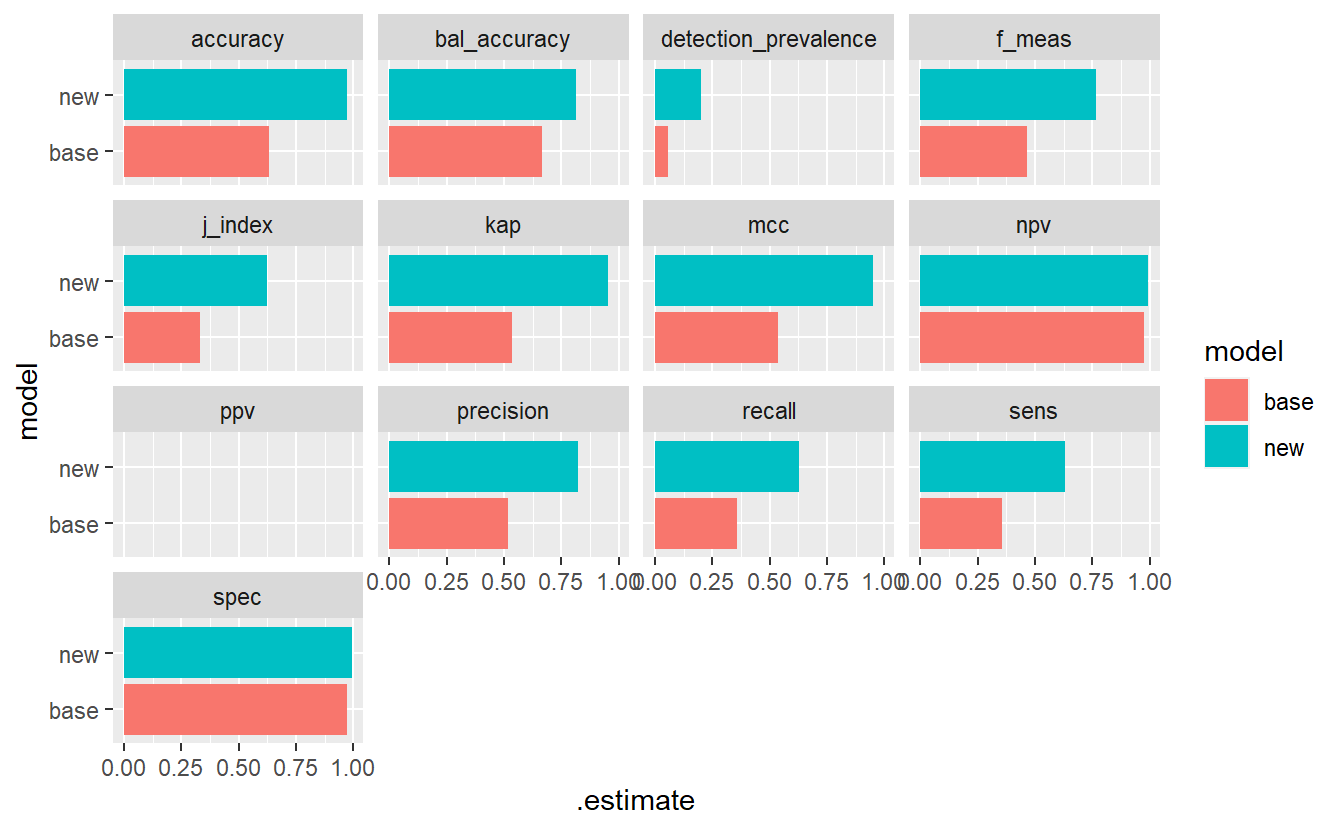Model Evaluation Metrics - base Vs new model

Let’s make another pass at this, this time we will group together

Code_Table_Factor_3 <- Code_Table_Factor %>%
mutate(Code_Description_3 = case_when(
Code %in% c(33, 34, 35) ~ "insulin dose",
Code %in% c(58, 60, 62, 64, 59, 61, 63) ~ "pre / post meal",
Code %in% c(66, 67, 68, 65, 69, 70, 71) ~ "meal ingestion / Hypoglycemic / exercise activity")) %>%
mutate(Code_Description_3 = factor(Code_Description_3)) %>%
select(Code, Code_Description_3)

Code_Table_Factor_3
#> # A tibble: 17 x 2
#>    Code Code_Description_3
#>   <dbl> <fct>
#> 1    33 insulin dose
#> 2    34 insulin dose
#> 3    35 insulin dose
#> 4    58 pre / post meal
#> 5    59 pre / post meal
#> 6    60 pre / post meal
#> # ... with 11 more rows
DATA.train_3 <- DATA.train %>% left_join(Code_Table_Factor_3)
#> Joining, by = "Code"

rf_model_3 <- randomForest(Code_Description_3 ~ Date_Time + Value_Num,
data = DATA.train_3,
ntree = 659,
importance = TRUE)
rm(DATA.train_3)
better_rand_forest_errors(rf_model_3)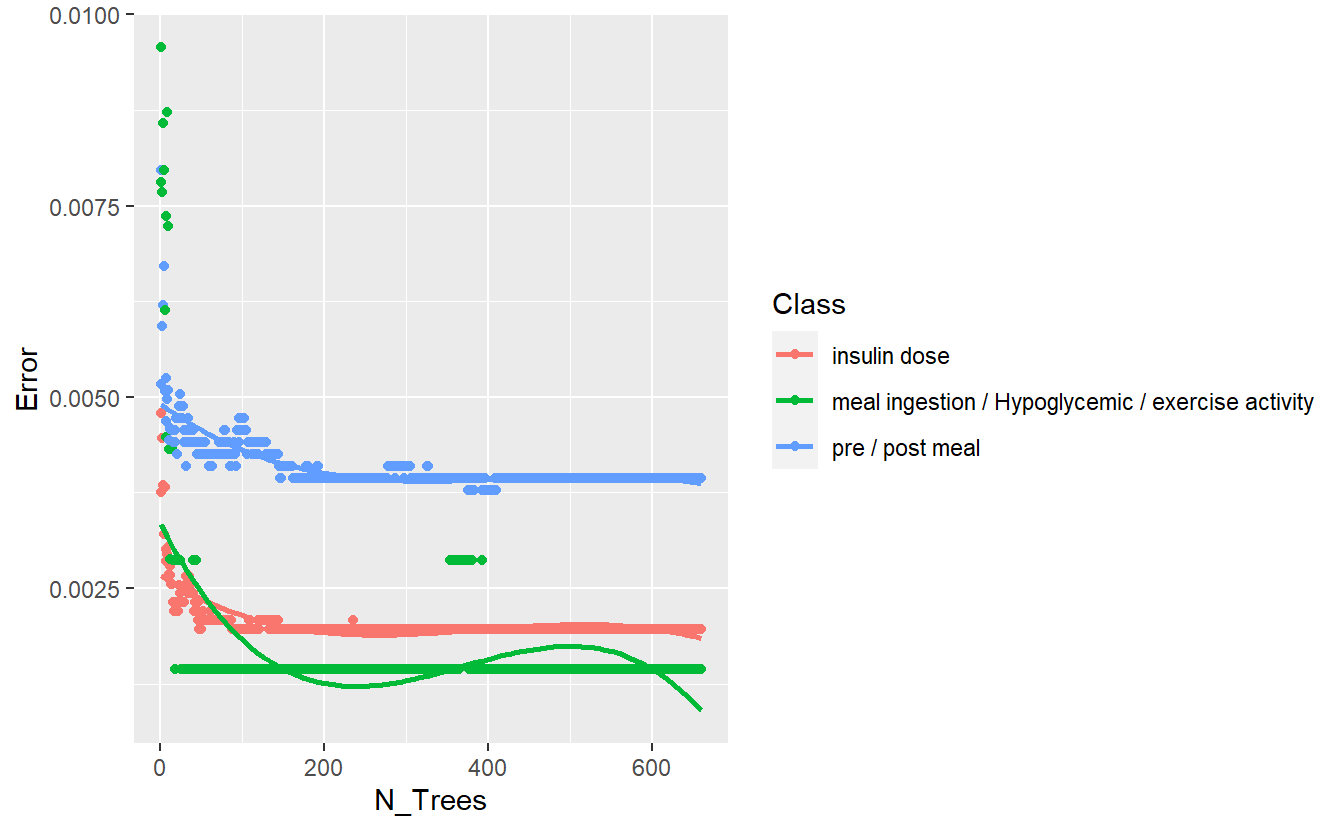Training Errors of Final Model

DATA.test.Scored3 <- DATA.test %>%
left_join(Code_Table_Factor_3) %>%
mutate(model = 'final')
#> Joining, by = "Code"

DATA.test.Scored3$pred <- predict(rf_model_3, DATA.test.Scored3 , 'response') conf_mat.DATA.test.Scored3 <- DATA.test.Scored3 %>% conf_mat(truth = Code_Description_3, pred) conf_mat.DATA.test.Scored3 %>% autoplot('heatmap')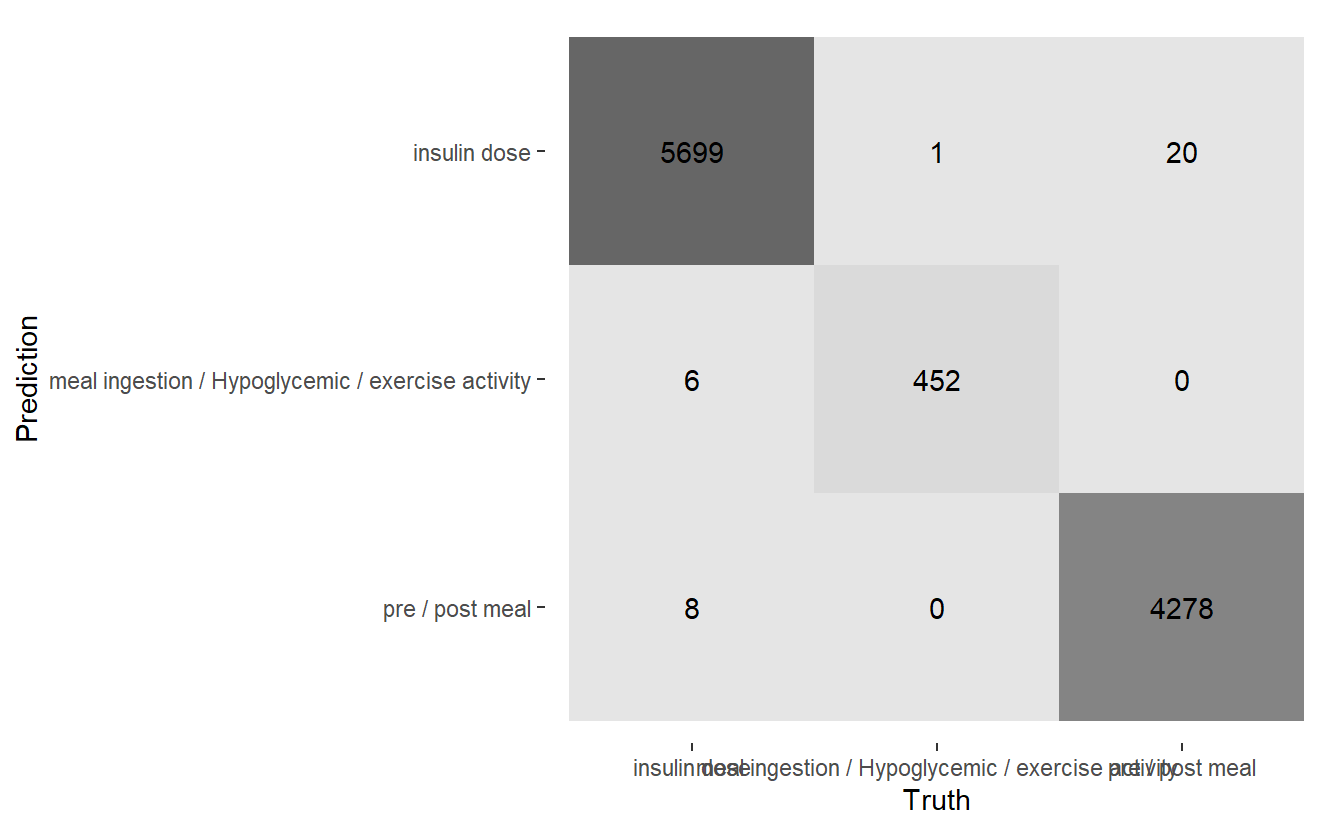Heatmap Confusion Matrix of Final Model saveRDS(rf_model_3, 'DATA/Part_5/models/rf_model_3.RDS') rm(rf_model_3) ## 13.12 Extract More Data library('ggplot2') library('ggdendro') library('tree') #> Registered S3 method overwritten by 'tree': #> method from #> print.tree cli #> #> Attaching package: 'tree' #> The following object is masked from 'package:igraph': #> #> tree tree_1 <- tree::tree(Code_Factor ~ Date_Time + Value_Num, data = DATA.train) #> Warning in tree::tree(Code_Factor ~ Date_Time + Value_Num, data = DATA.train): #> NAs introduced by coercion plot_dendro_tree <- function(model){ tree_data <- dendro_data(model) p <- segment(tree_data) %>% ggplot() + geom_segment(aes(x = x, y = y, xend = xend, yend = yend, size = n), colour = "blue", alpha = 0.5) + scale_size("n") + geom_text(data = label(tree_data), aes(x = x, y = y, label = label), vjust = -0.5, size = 3) + geom_text(data = leaf_label(tree_data), aes(x = x, y = y, label = label), vjust = 0.5, size = 2) + theme_dendro() p } plot_dendro_tree(tree_1) tree_2 <- tree::tree(Code_Factor ~ Date_Time + Value_Num + h + day + month , data = DATA.train_2 %>% mutate(h = factor(lubridate::hour(Date_Time))) %>% select(Code_Factor, Date_Time, Value_Num, h) %>% mutate(day = lubridate::day(Date_Time)) %>% mutate(month = month(Date_Time, label = TRUE)) ) #> Warning in tree::tree(Code_Factor ~ Date_Time + Value_Num + h + day + month, : #> NAs introduced by coercion plot_dendro_tree(tree_2)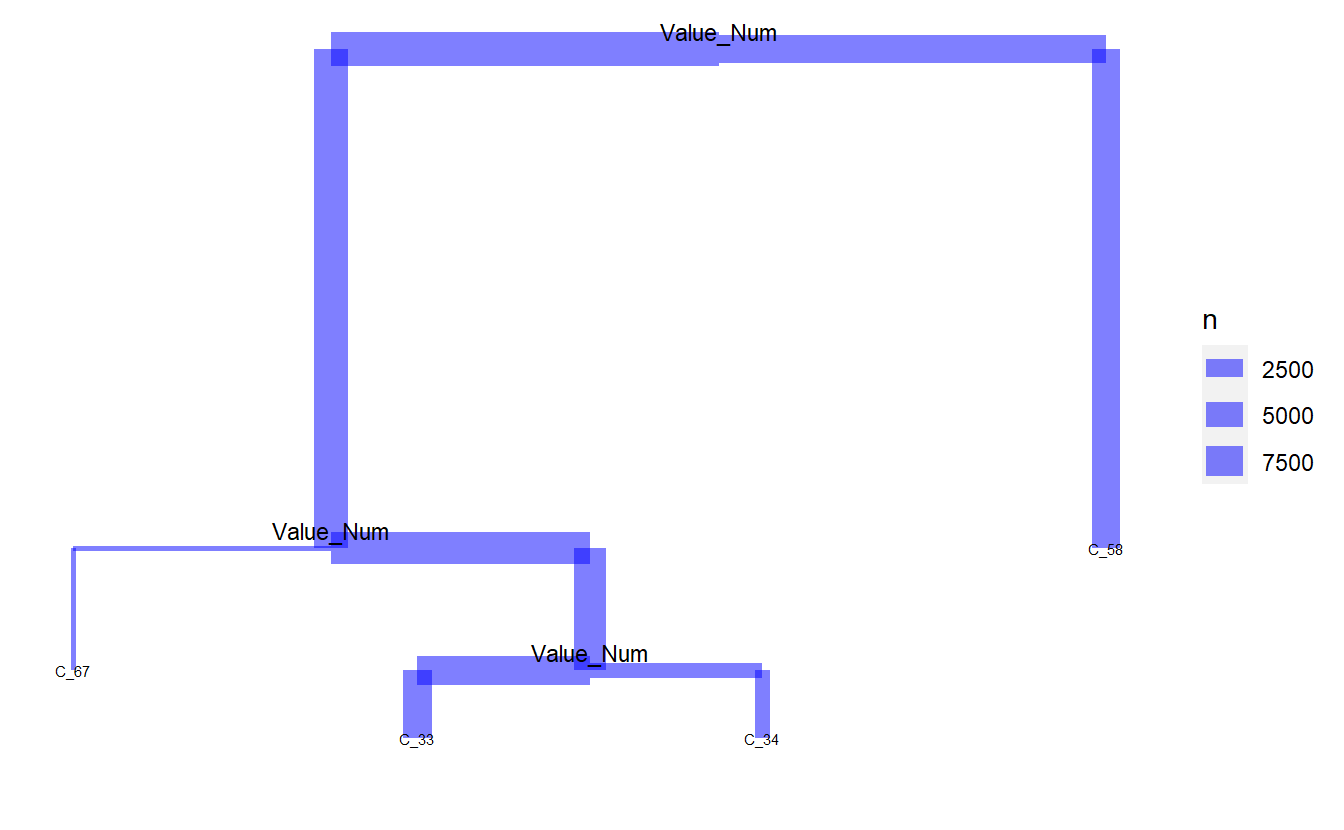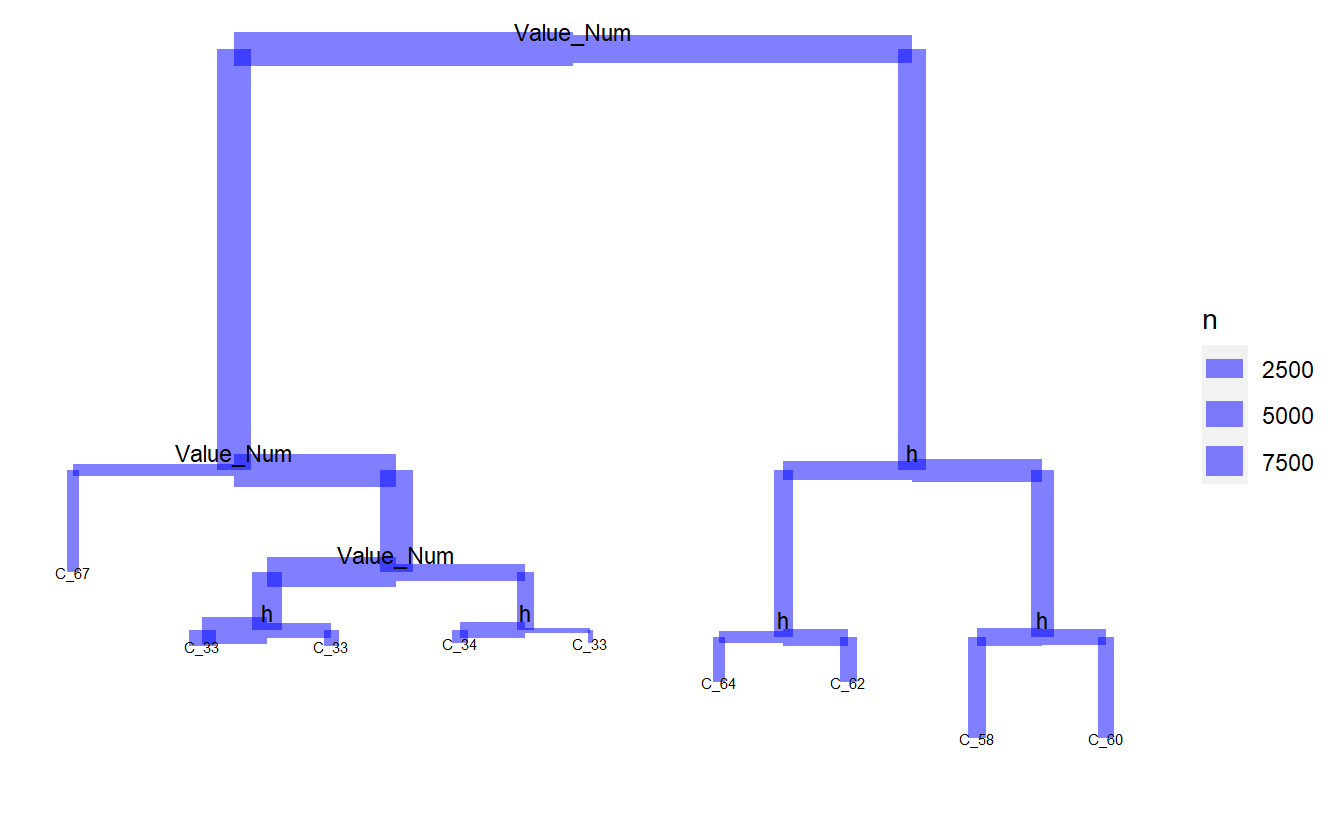rf_model_4 <- randomForest(Code_Description_2 ~ Date_Time + Value_Num + h + day + month, data = DATA.train_2 %>% mutate(h = factor(lubridate::hour(Date_Time))) %>% select(Code_Description_2, Code_Factor, Date_Time, Value_Num, h) %>% mutate(day = lubridate::day(Date_Time)) %>% mutate(month = month(Date_Time, label = TRUE)), ntree = 659, importance = TRUE) better_rand_forest_errors(rf_model_4)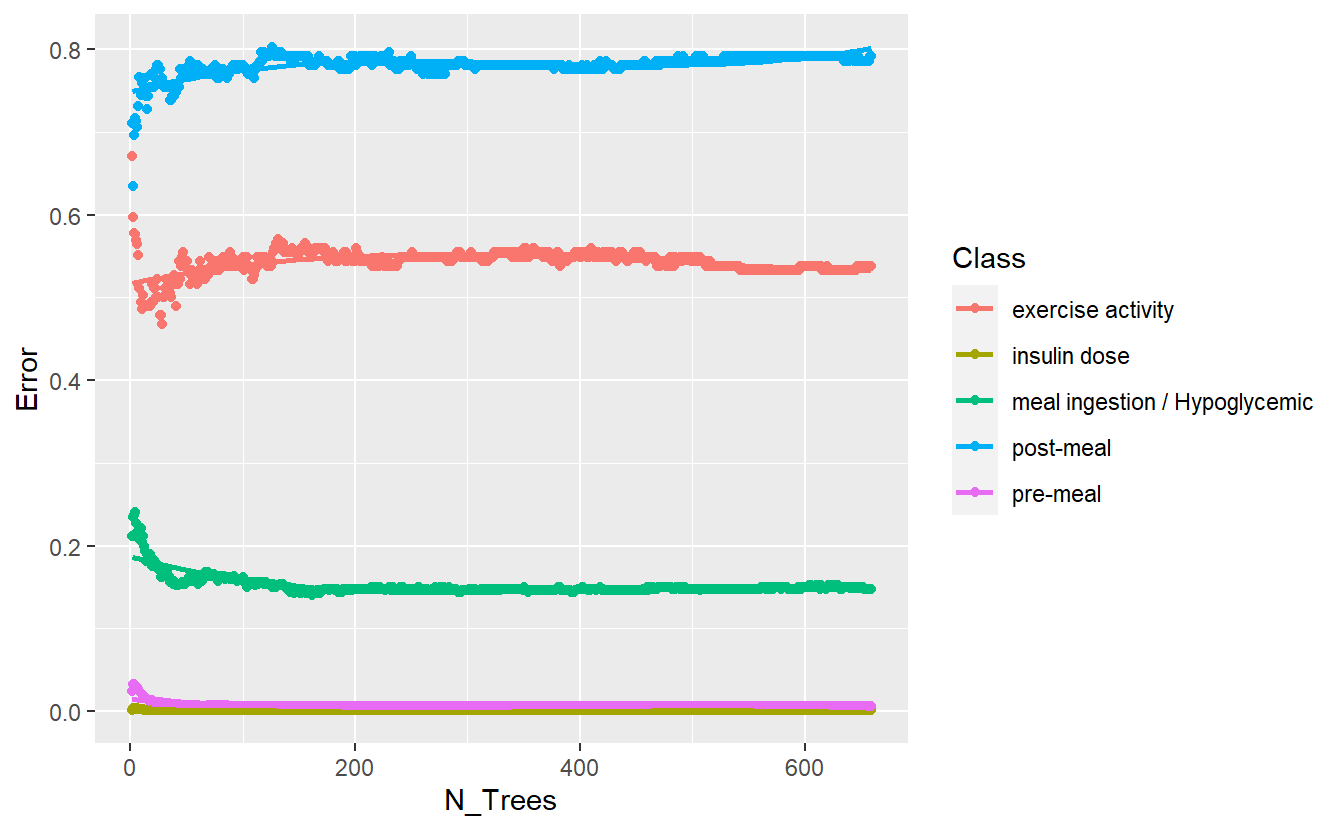DATA.test.Scored4 <- DATA.test %>% left_join(Code_Table_Factor_2) %>% mutate(model = 'final') %>% mutate(h = factor(lubridate::hour(Date_Time))) %>% mutate(day = lubridate::day(Date_Time)) %>% mutate(month = month(Date_Time, label = TRUE)) %>% select(Code_Description_2, Code_Factor, Date_Time, Value_Num, day, month, h) #> Joining, by = "Code" DATA.test.Scored4$pred <- predict(rf_model_4, DATA.test.Scored4 , 'response')

conf_mat.DATA.test.Scored4 <- DATA.test.Scored4 %>%
conf_mat(truth = Code_Description_2, pred)
slim <- conf_mat.DATA.test.Scored3 %>% summary() %>% mutate(model = 'slim')
final <- conf_mat.DATA.test.Scored4 %>% summary() %>% mutate(model = 'final')

comp_f <- bind_rows(comp,
slim,
final)

comp_f %>%
ggplot(aes(x=model, y=.estimate, fill = model)) +
geom_bar(stat='identity', position = 'dodge') +
coord_flip() +
facet_wrap(.metric ~ .)
#> Warning: Removed 2 rows containing missing values (geom_bar).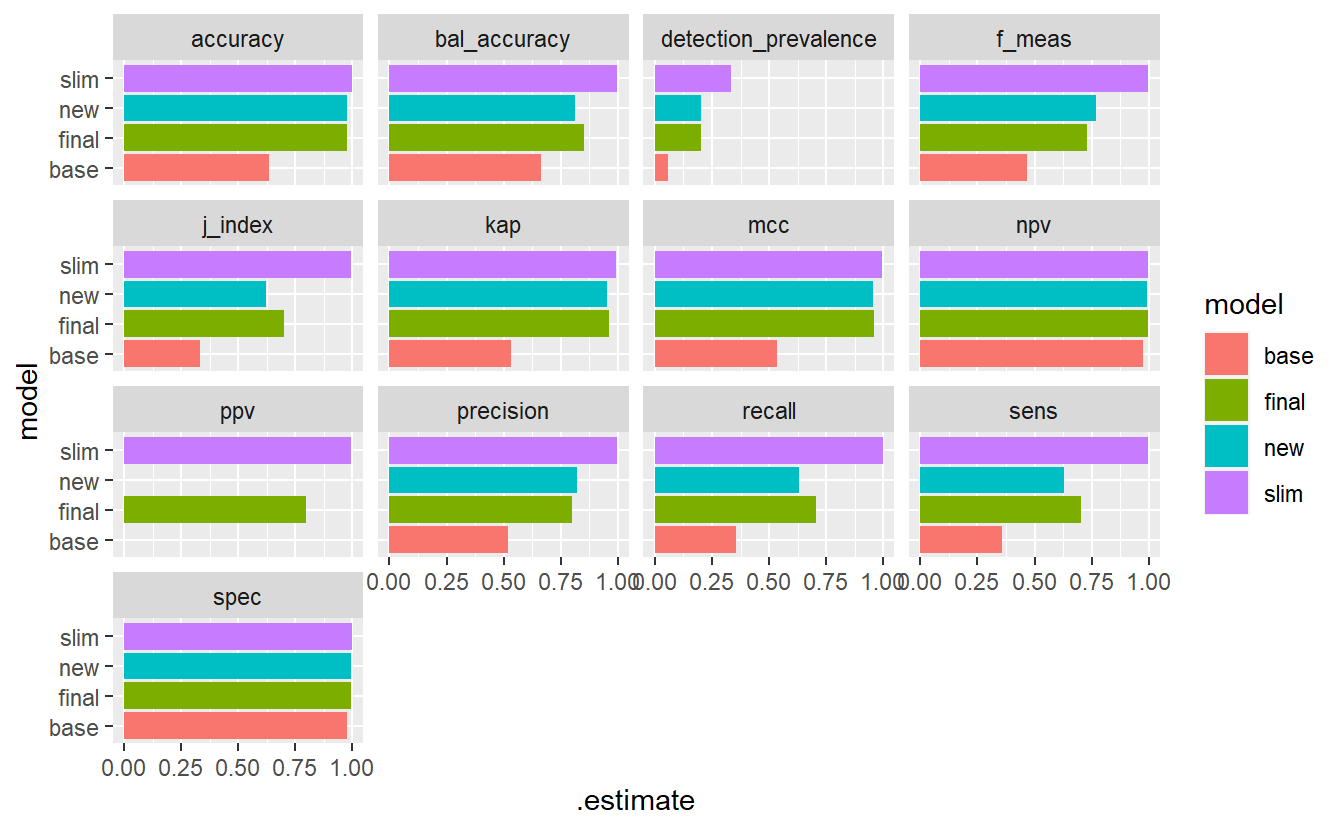Model Evaluation Metrics - base Vs new model Vs Final

## 13.13 Predict UnKnown Data

keep = c('UnKnown_Measurements','rf_model','rf_model_2','rf_model_3','rf_model_4','keep')

rm(list = setdiff(ls(), keep))

rf_model_3 <- readRDS('DATA/Part_5/models/rf_model_3.RDS')
UnKnown_Measurements$pred_base <- predict(rf_model, UnKnown_Measurements , 'response') UnKnown_Measurements$pred_new <- predict(rf_model_2, UnKnown_Measurements , 'response')
UnKnown_Measurements$pred_slim <- predict(rf_model_3, UnKnown_Measurements , 'response') UnKnown_Measurements <- UnKnown_Measurements %>% mutate(h = factor(lubridate::hour(Date_Time))) %>% mutate(day = lubridate::day(Date_Time)) %>% mutate(month = month(Date_Time, label = TRUE)) UnKnown_Measurements$pred_final <- predict(rf_model_4, UnKnown_Measurements, 'response')
UnKnown_Measurements %>%
ggplot(aes(x=Date_Time, y=Value_Num, color=pred_base)) +
geom_point()
#> Warning: Removed 86 rows containing missing values (geom_point).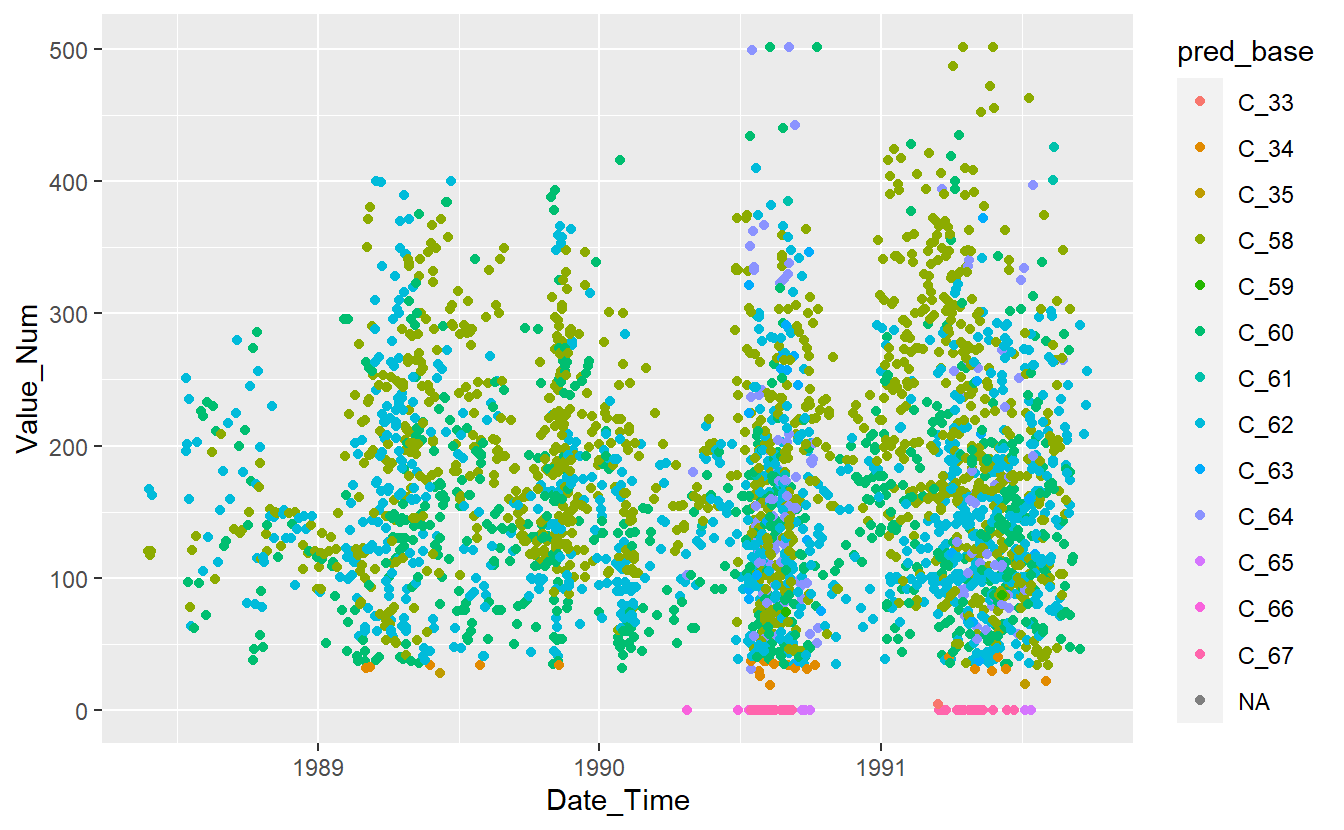Preject UnKnown Data with Base Model

UnKnown_Measurements %>%
ggplot(aes(x=Date_Time, y=Value_Num, color=pred_new)) +
geom_point()
#> Warning: Removed 86 rows containing missing values (geom_point).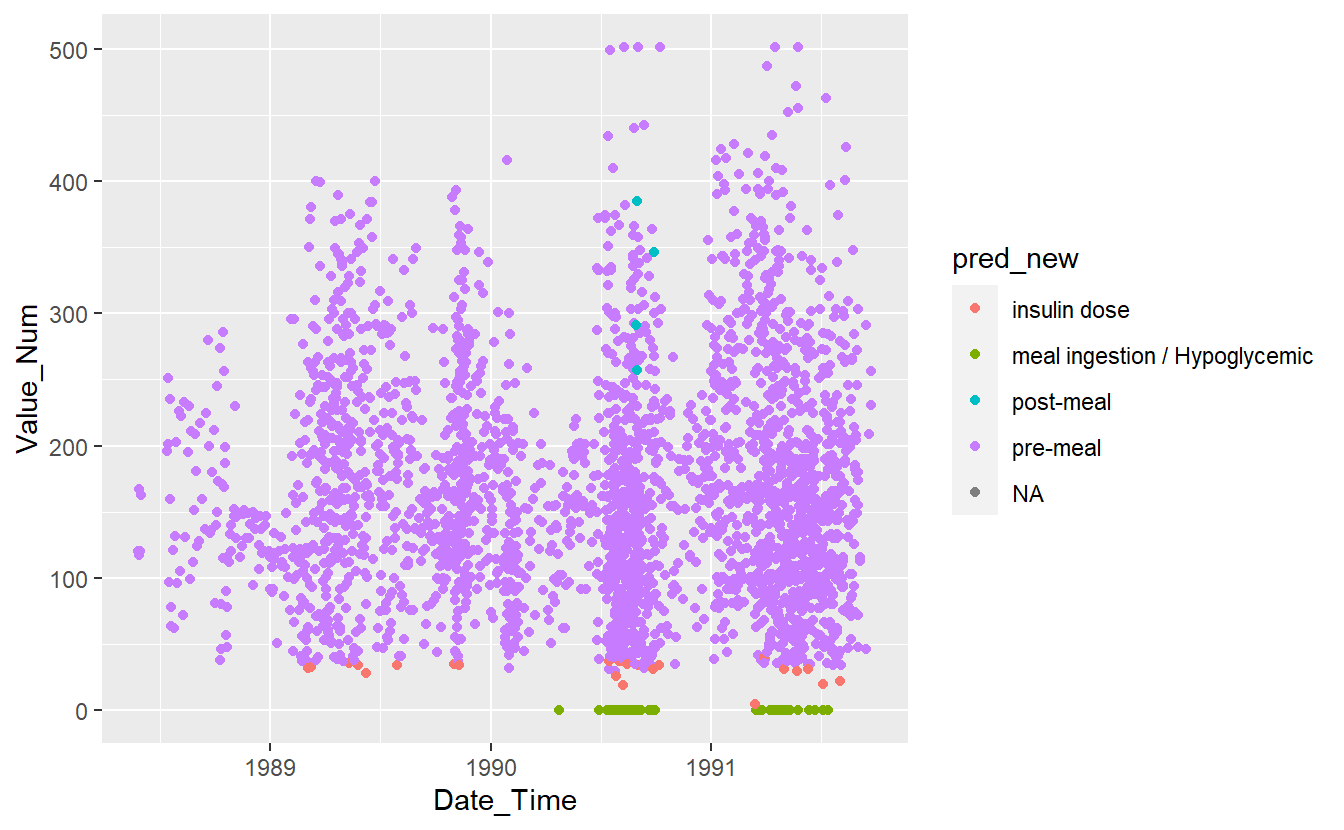Preject UnKnown Data with New Model

UnKnown_Measurements %>%
ggplot(aes(x=Date_Time, y=Value_Num, color=pred_slim)) +
geom_point()
#> Warning: Removed 86 rows containing missing values (geom_point).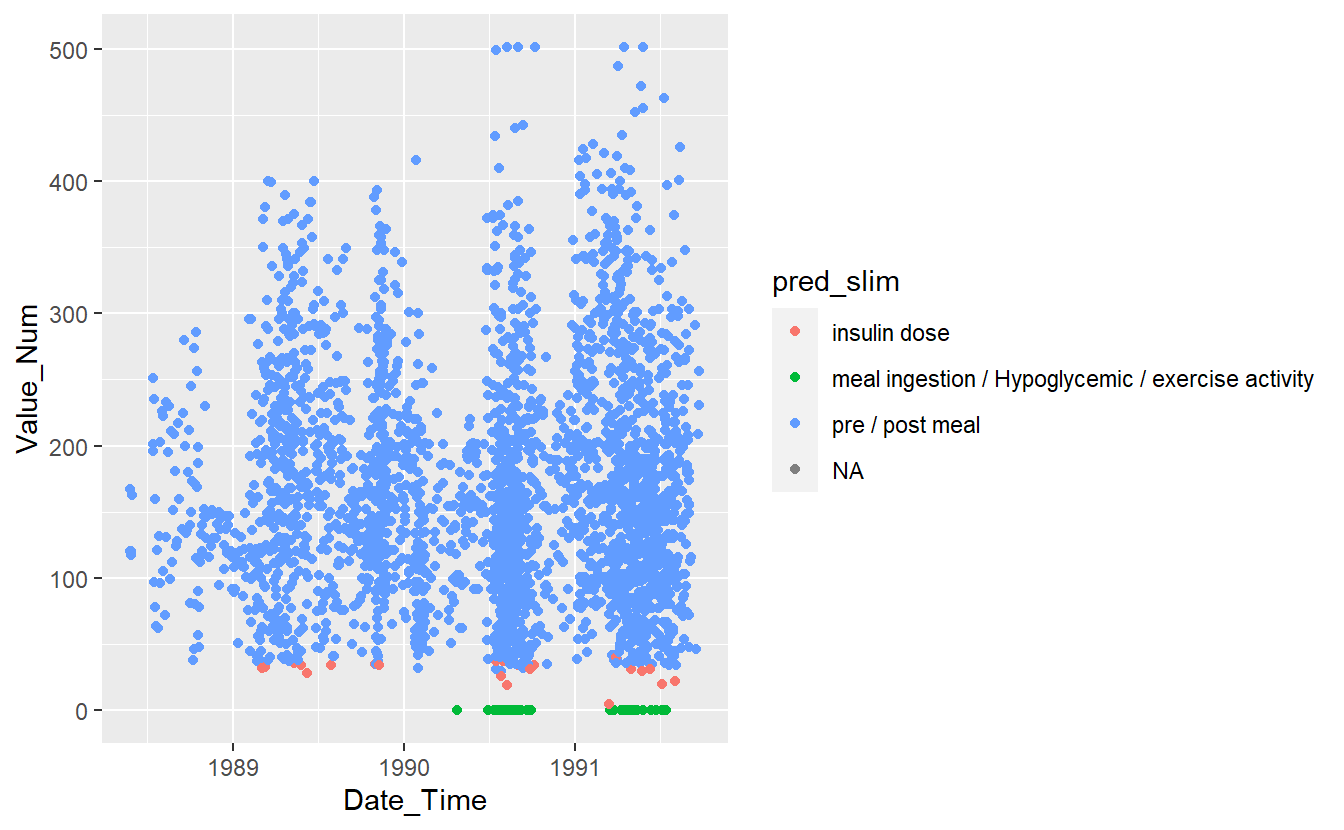Preject UnKnown Data with slim Model

UnKnown_Measurements %>%
ggplot(aes(x=Date_Time, y=Value_Num, color=pred_final)) +
geom_point()
#> Warning: Removed 86 rows containing missing values (geom_point).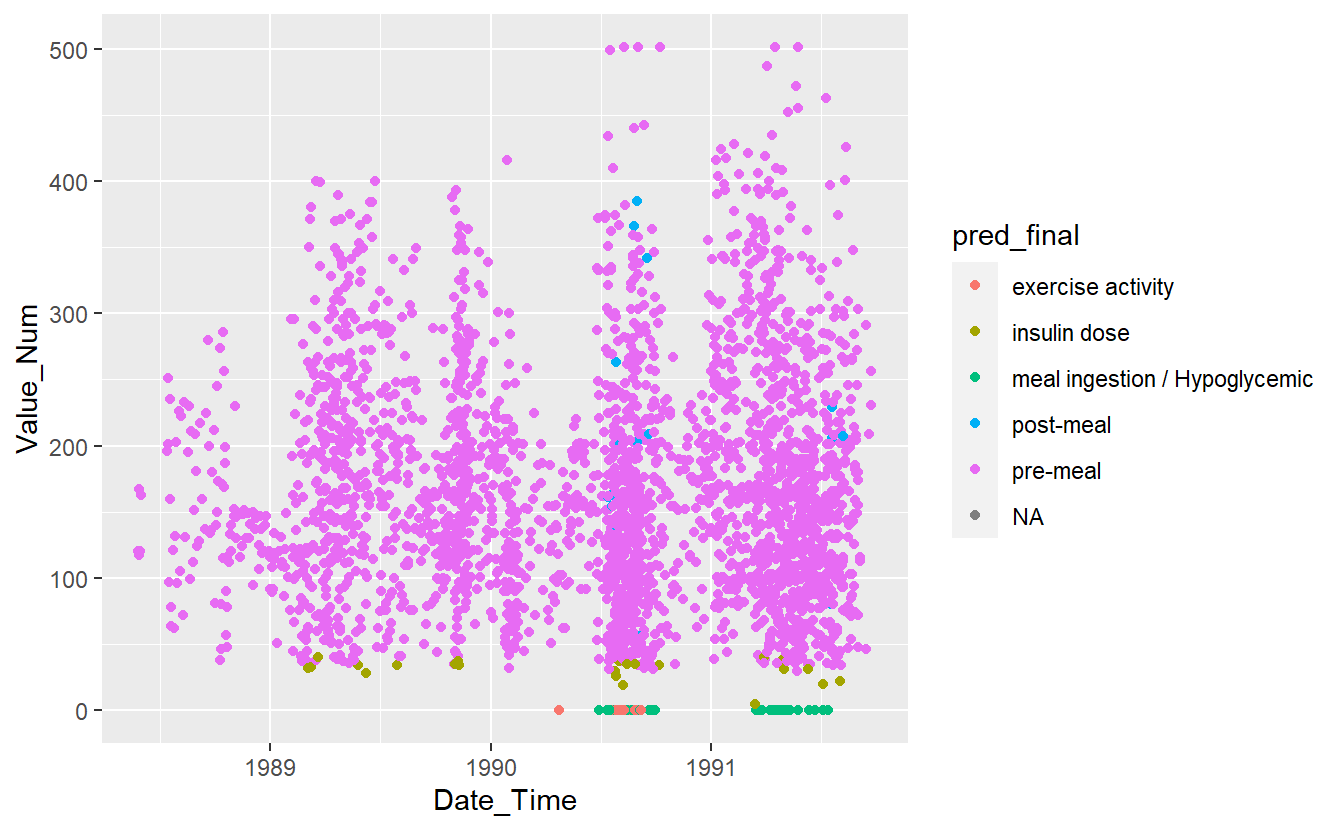Preject UnKnown Data with Final Model